Chapter

6

Nonparametric Methods

Purposes of Nonparametric Methods:

Nonparametric methods are uniquely useful for testing nominal (categorical) and ordinal (ordered) scaled data--situations where parametric tests are not generally available.  An important second use is when an underlying assumption for a parametric method has been violated.  In this case, the interval/ratio scale data can be easily transformed into ordinal scale data and the counterpart nonparametric method can be used.

Inferential and Descriptive Statistics: The nonparametric methods described in this chapter are used for both inferential and descriptive statistics.  Inferential statistics use data to draw inferences (i.e., derive conclusions) or to make predictions.  In this chapter, nonparametric inferential statistical methods are used to draw conclusions about one or more populations from which the data samples have been taken.  Descriptive statistics aren’t used to make predictions but to describe the data.  This is often best done using graphical methods.

Examples: An analyst or engineer might be interested to assess the evidence regarding:
1.   The difference between the mean/median accident rates of several marked and unmarked crosswalks (when parametric Student’s t test is invalid because sample distributions are not normal).
2.   The differences between the absolute average errors between two types of models for forecasting traffic flow (when analysis of variance is invalid because distribution of errors is not normal).
3.   The relationship between the airport site evaluation ordinal rankings of two sets of judges, i.e., citizens and airport professionals.
4.   The differences between neighborhood districts in their use of a regional mall for purposes of planning transit routes.
5.   The comparison of accidents before and during roadway construction to investigate if factors such as roadway grade, day of week, weather, etc. have an impact on the differences.
6.   The association between ordinal variables, e.g., area type and speed limit, to eliminate intercorrelated dependent variables for estimating models that predict the number of utility pole accidents.
7.   The relative goodness of fit of possible predictive models to the observed data for expected accident rates for rail-highway crossings.
8.  The relative goodness of fit of hypothetical probability distributions, e.g., lognormal and Weibull, to actual air quality data with the intent of using the distributions to predict the number of days with observed ozone and carbon monoxide concentrations exceeds National Ambient Air Quality Standards.

Basic Assumptions/Requirements; Nonparametric Vs. Parametric Methods:

Nonparametric methods are contrasted to those that are parametric.  A generally accepted description of a parametric method is one that makes specific assumptions with regard to one or more of the population parameters that characterize the underlying distribution(s) for which the test is employed.  In other words, it a test that assumes the population distribution has a particular form (e.g., a normal distribution) and involves hypotheses about population parameters.  Nonparametric tests do not make these kinds of assumptions about the underlying distribution(s) (but some assumptions are made and must be understood).

Nonparametric methods use approximate solutions to exact problems, while parametric methods use exact solutions to approximate problems.
W.J. Conover

Remember the overarching purpose for employing a statistical test is to provide a means for measuring the amount of subjectivity that goes into a researcher’s conclusions.  This is done by setting up a theoretical model for an experiment.  Laws of probability are applied to this model to determine what the chances (probabilities) are for the various outcomes of the experiment assuming chance alone determines the outcome of the experiment.  Thus, the researcher has an objective basis to decide if the actual outcome from his or her experiment were the results of the treatments applied or if they could have occurred just as easily by chance alone, i.e., with no treatment at all.

When the researcher has described an appropriate theoretical model for the experiment (often not a trivial task), the next task is to find the probabilities associated with the model.  Many reasonable models have been developed for which no probability solutions have ever been found.  To overcome this, statisticians often change a model slightly in order to be able to solve the probabilities with the hope that the change doesn’t render the model unrealistic. These changes are usually “slight” to try to minimize the impacts, if possible.  Thus, statisticians can obtain exact solutions for these “approximate problems.”  This body of statistics is called parametric statistics and includes such well-known tests as the “t test” (using the t distribution) and the F test (using the F distribution) as well as others.

Nonparametric testing takes a different approach, which involves making few, if any, changes in the model itself. Because the exact probabilities can’t be determined for the model, simpler, less sophisticated methods are used to find the probabilities--or at least a good approximation of the probabilities.  Thus, nonparametric methods use approximate solutions to exact problems, while parametric methods use exact solutions to approximate problems.

Statisticians disagree about which methods are parametric and which are nonparametric.  Such disagreements are beyond the scope of this discussion.  Perhaps one of the easiest definitions to understand, as well as being fairly broad, is one proposed by W.J. Conover (1999, p.118).

Definition:  A statistical method is nonparametric if it satisfies at least one of the following criteria:

1.  The method may be used on data with a nominal scale of measurement.

2.  The method may be used on data with an ordinal scale of measurement.

3. The method may be used on data with an interval or ratio scale of measurement, where the distribution function of the random variable producing the data are unspecified (or specified except for an infinite number of unknown parameters

Herein lies a primary usefulness of nonparametric tests: for testing nominal and ordinal scale data.  There is debate about using nonparametric tests on interval and ratio scale data.  There is general agreement among researchers that if there is no reason to believe that one or more of the assumptions of a parametric test has been violated, then the appropriate parametric test should be used to evaluate the data.  However, if one or more of the assumptions have been violated, then some (but not all) statisticians advocate transforming the data into a format that is compatible with the appropriate nonparametric test.  This is based on the understanding that parametric tests generally provide a more powerful test of an alternative hypothesis than their nonparametric counterparts; but if one or more of the underlying parametric test assumptions is violated, the power advantage may be negated.

The researcher should not spend too much time worrying about which test to use for a specific experiment.  In almost all cases, both tests applied to the same data will lead to identical or similar conclusions. If conflicting results occur, the researcher would be well advised to conduct additional experiments to arrive at a conclusion, rather than simply pick one or the other method as being “correct.”

Examples of Nonparametric Methods:

Environment

Chock, David P. and Paul S. Sluchak. (1986). Estimating Extreme Values of Air Quality Data Using Different Fitted Distributions.  Atmospheric Environment, V.20, N.5, pp. 989-993. Pergamon Press Ltd.  (Kolmogorov-Smirnov Type Goodness of Fit (GOF) Tests and Chi-Square GOF Test)

Safety

Ardeshir, Faghri, Demetsky Michael J. (1987). Comparison of Formulae for Predicting Rail-Highway Crossing Hazards. Transportation Research Record #1114 pp. 152-155. National Academy of Sciences.  (Chi-Square Goodness of Fit Test)

Davis, Gary A. and Yihong Gao. (1993). Statistical Methods to Support Induced Exposure Analyses of Traffic Accident Data. Transportation Research Record #1401 pp. 43-49. National Academy of Sciences.  (Chi-Square Test for Independence)

Gibby, A. Reed, Janice Stites, Glen S. Thurgood, and Thomas C. Ferrara. (1994). Evaluation of Marked and Unmarked Crosswalks at Intersections in California. Federal Highway Administration, FHWA/CA/TO-94-1 and California Department of Transportation (Caltrans) CPWS 94-02, 66 pages.  (Mann-Whitney Test for Two Independent Samples)

Hall, J. W. and V. M. Lorenz.(1989). Characteristics of Construction-Zone Accidents. Transportation Research Record #1230 pp. 20-27. National Academy of Sciences.  (Chi-Square Test for Independence)

Kullback, S. and John C. Keegel. (1985). Red Turn Arrow: An Information-Theoretic Evaluation. Journal of Transportation Engineering, V.111, N.4, July, pp. 441-452. American Society of Civil Engineers.  (Inappropriate use of Chi-Square Test for Independence)

Zegeer, Charles V., and Martin R. Parker Jr. (1984). Effect of Traffic and Roadway Features on Utility Pole Accidents. Transportation Research Record #970 pp. 65-76. National Academy of Sciences.  (Kendall’s Tau Measure of Association for Ordinal Data)

Traffic

Smith, Brian L. and Michael J. Demetsky. (1997). Traffic Flow Forecasting: Comparison of Modeling Approaches. Journal of Transportation Engineering, V.123, N.4, July/August, pp. 261-266. American Society of Civil Engineering.  (Wilcoxon Matched-Pairs Signed-Ranks Test for Two Dependent Samples)

Transit

Ross, Thomas J. and Eugene M. Wilson. (1977). Activity Based Transit Routing. Transportation Engineering Journal, V.103, N.TE5, September, pp. 565-573. American Society of Civil Engineers.  (Chi-Square Test for Independence)

Planning

Jarvis, John J., V. Ed Unger, Charles C. Schimpeler, and Joseph C. Corradino. (1976). Multiple Criteria Theory and Airport Site Evaluation. Journal of Urban Planning and Development Division, V.102, N.UP1, August, pp. 187-197.  American Society of Civil Engineers.  (Kendall’s Tau Measure of Association for Ordinal Data

Nonparametric References:

·         W. J. Conover.  “Practical Nonparametric Statistics.”  Third Edition, John Wiley & Sons, New York, 1999.

·         W. J. Conover.  “Practical Nonparametric Statistics.”  Second Edition, John Wiley & Sons, New York, 1980.

·         Richard A. Johnson.  “Miller & Freund’s Probability and Statistics For Engineers.”  Prentice Hall, Englewood Cliffs, New Jersey, 1994.

·         Douglas C. Montgomery.  “Design and Analysis of Experiments.”  Fourth Edition, John Wiley & Sons, New York, 1997.

·         David J. Sheskin.  “Handbook of Parametric and Nonparametric Statistical Procedures.”  CRC Press, New York, 1997.

Purpose of Graphical Methods:

Graphical methods can be thought of as a way to obtain a “first look” at a group of data.  It does not provide a definitive interpretation of the data, but can lead to an intuitive “feel” for the data.  However, this “first look” can be deceiving because the human mind wants to classify everything it views based on something it already knows.  This can lead to an erroneous first impression that can be hard for a researcher to dismiss; even as evidence mounts that it may be wrong.  This is human nature at work, wanting to be consistent.  It is important for a researcher to be aware of this need for consistency, which can cloud objectivity.

The parable of the three blind men encountering an elephant for the first time effectively illustrates this human tendency to make a judgement based on past experience and then to “stop looking” for more clues.  In the parable, the three blind men approached the elephant together.  The first man touches the elephant’s trunk and thoroughly investigates it.  He backs away and declares “the elephant is exactly like a hollow log, only alive and more flexible.”  The second man touches one of the elephant’s massive feet and explores it in many places.  He backs away and declares, “the elephant is very much like a large tree growing from the ground, only its bark is warm to the touch.”  The last man grasps the elephant’s tail and immediately declares “the elephant is simply a snake that hangs from something, probably a tree.”

All of the information gathered by the three blind men is important and pooled might provide a “good” model of an elephant.  Graphical methods should be thought of as a single blind investigation.  The data viewed is not wrong, but a conclusion drawn solely from them can be wrong.  Keep in mind that the purpose of graphical methods is simply to get a first look at the data without drawing conclusions.  It can, however, lead to hypotheses that guide further investigation.

Examples: An analyst or engineer might be interested in exploring data to:
1.   See if potential relationships, either linear or curvilinear, exist between a variable of interest whose values may depend on several other variables.
2.   See if interactions (relationships) may exist between a variable and two other variables.

Scatter Plots, Pairwise Scatter Plots, and Brush and Spin

Scatter plots are the workhorses of graphical methods.  In two dimensions, the data points are simply plotted by specifying two variables to form the axes.  This technique is often used to develop a first impression about the relationship between two variables.  For example, the variables in the two scatter plots below appear to have quite different relationships.

As a first look, X1 appears to have a linear relationship with Y1 while X2 appears to have a non-linear relationship.  Hypothesizing such apparent relationships are useful in selecting a preliminary model type and relationship.  Many statistical software packages make it easy for the user to study such relationships among all the variables in the data.  This method is typically called “pairwise” scatter plots but other terms are also used.  The three variables explored in the previous scatter plots are used again to plot the pairwise scatter plots shown below.

As a first look, X1 appears to have a linear relationship with Y1 while X2 appears to have a non-linear relationship.  Hypothesizing such apparent relationships are useful in selecting a preliminary model type and relationship.  Many statistical software packages make it easy for the user to study such relationships among all the variables in the data.  This method is typically called “pairwise” scatter plots but other terms are also used.  The three variables explored in the previous scatter plots are used again to plot the pairwise scatter plots shown below.Figure 17: Scatter Plots of Y1 vs. X1, and Y1 vs. X2

By using pairwise scatter plots, the relationships among all the variables may be explored with a single plot.  However, as the number of variables increases, using a single plot reduces the individual plots too small to be useful.  In this case, the variables can be plotted in subsets.Figure 18: Pair-wise Scatter Plots for Y1, X2, and X2

The scatter plots described so far are two-dimensional.  Three-dimensional methods are also available and are often used in exploring three variables from the data.  The data displayed in this fashion form as a “point cloud” using three axes.  The more powerful statistical software packages allow these point clouds to be rotated or spun around any axis.  This gives hints as to any interactions between two variables as it affects a third variable.  Another feature often available is a brush technique, which allows the user to select specific points to examine.  These points then become bigger or of a different color than the remainder of the data, which allows the user to see their spatial relationship within the point cloud.  Usually a pairwise scatter plot is simultaneously displayed on the same screen and the selected points are also highlighted in each of these scatter plots.  This allows the user to study individual points or clusters of points.  Examples that are useful to explore are outliers and clusters of points that are seemingly isolated from the rest of the data points.

Three Dimensional Graphics: Contours and Response Surfaces

Three-dimensional graphics allow the user to investigate three variables simultaneously.  Two plots that are often used are the contour plot and the surface plot.  Examples using the three variables explored previously in the scatter plots are shown below.  The contour plot shows the isobars of Y1 for the range of values of X1 and X2 contained within the plot.  The surface plot fits a “wire mesh” through the X1, X2, and Y1 coordinates to give the user a perspective of the surface created by the data.  In both of these graphs the values between the data values are interpolated.  The user is cautioned that these interpolations are not validated and a “smooth” transition from one point to the next may not be a true representation of the data.  Like all graphical methods, these should be used only to obtain a first look at the data, and when appropriate, aid in developing preliminary hypotheses--along with other available information--for modeling the data.Figure 19: Contour and Surface Plots for Variables Y1, X1, and X2

Purpose of Descriptive Techniques:

One way to better understand what descriptive statistics are is to first describe inferential statistics.  If the data being investigated are a sample that is representative of a larger population, important conclusions about that population can often be inferred from analysis of the sample.  This is usually called inferential statistics, although sometimes it may be called interpretative statistics.  Because such inferences cannot be absolutely certain, probability is used in stating conclusions.  This is an inductive process and is used in the sections of this chapter that follow this one.

Descriptive techniques are discussed in this section and are another important first step in exploring data.  The purpose of these techniques is to describe and analyze a sample without drawing any conclusions or inferences about a larger group from which it may have been drawn.  This is a deductive process and is quite useful in developing an understanding of the data.  Occasionally this is all that is needed, but often it is a preliminary step toward modeling the data.

Examples: An analyst or engineer might be interested in exploring data to:
1.   Quantitatively assess its dispersion, i.e., to determine whether ifs values are bunched tightly about some central value or not.
2.   Quantitatively assess all the values of the data, perhaps to qualitatively examine a suspicion that the data may have some distribution of values that is similar to something the investigator has seen before.
3.   Quantitatively compare two or more sets of data in order to qualitatively examine what potential similarities or significant differences might be present.

Basic Descriptive Statistics of Data:  Mean, Median, and Quartiles

Almost all general statistical reference books and textbooks thoroughly describe these types of descriptive statistics, one such reference is Johnson (1994).  Briefly the mean is typically the arithmetic mean, which is simply the average of all the numbers in the data sample.  The median is the middle value when all the values in a data variable are ranked in order such that ties are ranked one above the other rather than together.  The median is also the second quartile of the data variable.  Quartiles are the dividing points that separate the data variable values into four equal parts.  The first or lower quartile has 1/4 or 25% of the ranked data variable values below its value.  The third or upper quartile has 3/4 or 75% of the values below it.  Some references reverse the first and third labels, so the upper and lower labels create less confusion.

Frequency Distributions, Variance, Standard Deviation, Histograms, and Boxplots

The variance and standard deviation are quantitative measures of dispersion, i.e., the spread of a distribution.  Histograms and Boxplots are graphical ways to view the dispersion.  A frequency distribution is simply a table that divides a set of observed data values into a suitable number of classes or categories, indicating the number of items belonging in each class.  This provides a useful summary of the location, shape and spread of the data values.  Consider the data used in the previous examples, which are provided in the following table.

Table 4: Observed Data Values

 Y1 X1 X2 5 25 25 6 28 28 7 36 36 8 41 41 9 44 44 10 53 43 11 54 39 12 61 35 13 66 26 14 72 21

In order to create a frequency distribution for a variable, appropriate classes must be selected for it.  Using both X1 and X2 as examples, classes of 0 to 10, 11 to 20, etc. are chosen and a frequency table constructed as shown below.

Table 5: Frequency Distribution of Observed Data

 Class Limits X1 X2 0 - 10 0 0 11-20 0 0 21-30 2 4 31-40 1 3 41-50 2 3 51-60 2 0 61-70 2 0 71-80 1 0 Totals 10 10 Variance 252.00 67.73 Standard Deviation 15.87 8.23

The variance and standard deviation are also shown in the table along with the frequency distribution.  These are measures of the spread of the data about the mean.  If all the values are bunched close to the mean, then the spread is “small.”  Likewise, the spread is large if all the values are scattered widely about their mean. A measure of the spread of data is useful to supplement the mean in describing the data.  If a set of numbers x1, x2, ... , xn has a mean xbar, the differences x1- xbar, x2- xbar,..., xn- xbar are called the deviations from the mean.  Because the sum of these deviations is always zero, an alternative approach is to square each deviation. The sample variance, s2, is essentially the average of the squared deviations from the mean.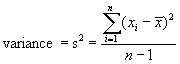By dividing the sum of squares by its degrees of freedom, n-1, an unbiased estimate of the population variance is obtained. Notice that s2 has the wrong units, i.e., not the same units as the variable itself.  To correct this, the standard deviation is defined as the square root of the variance, which has the same units as the data. Unlike the variance estimate, the estimated standard deviation is biased, where the bias becomes larger as sample sized become smaller.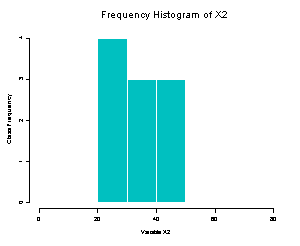.

Figure 20: Frequency Histograms of X1 and X2

While these quantitative descriptive statistics are useful, it is often valuable to provide graphical representations of them.  The frequency distributions can be shown graphically using frequency histograms as shown below for X1 and X2.

As can be seen from these two frequency histograms, X2 has a much smaller spread (standard deviation = 8.23) than does X1 (standard deviation = 15.87).  Another plot, the boxplot, also shows the quartiles of the frequency.  These are shown in Figure 21 for X1 and X2.

Different statistical software packages typically depict Boxplots in different ways, but the one shown here is typical.  Boxplots are particularly effective when several are placed side-by-side for comparison.  The shaded area indicates the middle half of the data.  The center line inside this shaded area is drawn at the median value.  The upper edge of the shaded area is the value of the upper quartile and the lower edge is the value of the lower quartile.  Lines extend from the shaded areas to the maximum and minimum values of the data indicated by horizontal lines.Figure 21: Boxplots of X1 and X2

Purposes of Ranking and Counting Methods:

The primary purpose of ranking and counting methods is hypothesis testing for ordinal scale data and for interval and ratio scale data without reliance on distribution assumptions.  Data may be nonnumeric or numeric.  If the data are nonnumeric but ranked as in ordinal-type data (e.g., low, medium, high), the methods in this section are often the most powerful ones available (Conover, 1999).  These methods are valid for continuous and discrete populations as well as mixtures of the two.

The hypotheses tested using these methods all involve medians.  While at first glance this may seem to be of limited usefulness, it is surprisingly versatile.  When the distributions of the random variables tested are assumed to be symmetric, the mean and the median are equal so these hypotheses also test means.  Usually the data, or statistics extracted from the data, are ranked from least to greatest.  Data may have many ties.  Two observations are said to be tied if they have the same value.

Early results in nonparametric statistics required the assumption of a continuous random variable in order for the tests based on ranks to be valid.  Research results reported by Conover (1999) and others have shown that the continuity assumption is not necessary.  It can be replaced by the trivial assumption that P(X = x) < 1 for each x.  It is unlikely that any sample will be taken from a population with only a single member.  Since this assumption is trivial, it is not listed for each test in this section, but it is what allows these tests to be valid for all types of populations: continuous, discrete, or mixtures of the two.

Examples: An analyst or engineer might be interested to assess the evidence regarding the difference between the mean/median values of:
1.   Accident rates of several marked and unmarked crosswalks (when parametric Student’s t test is invalid because sample distributions are not normal).
2.   The differences between the absolute average errors between two types of models for forecasting traffic flow (when analysis of variance is invalid because distribution of errors is not normal).

Ranking and Counting Methodology for Hypotheses About Medians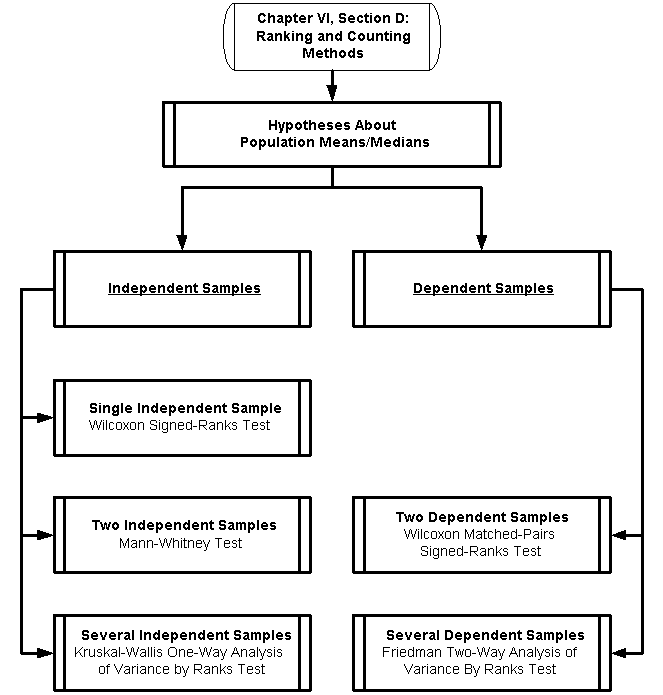Ranking and Counting Methodology for Hypotheses About Medians (and Means):

Are the samples independent or dependent?

Data collection is usually called sampling in statistical terms.  Sampling is the process of selecting some part of a population to observe so as to estimate something of interest about the whole population.  A population is the statistical term referring to the entire aggregate of individuals, items, measurements, things, numbers, etc. from which samples are drawn.  Two samples are said to be mutually independent if each sample is comprised of different subjects.  Dependent samples usually have the same subjects as in a comparative study, e.g., a before and after study or how a subject responds to two different situations (called treatments).

Hypotheses About Population Medians for Independent Samples

Wilcoxon Signed-Ranks Test - Hypothesis About Population Medians (Means) for a Single Independent Sample

This rank test was devised by F. Wilcoxon in 1945.  It is designed to test whether a particular sample came from a population with a specified median.  This test is similar to but more powerful than the classic sign test, which is not presented here.  The classic sign test is the oldest of all nonparametric tests, dating back to 1710, when it was used by J. Arbuthnott to compare the number of males born in London to the number of females born there.  The sign test is simpler to use than the more powerful nonparametric tests and is popular for that reason.  With today’s computer software packages, however, this is no longer a factor.

ASSUMPTIONS OF WILCOXON SIGNED-RANKS TEST FOR SINGLE INDEPENDENT SAMPLE

1)       The sample is a random sample.

2)       The measurement scale is at least interval.

3)       The underlying population distribution is symmetrical.  A distribution of a random variable X is symmetrical about a line having a value of x = c if the probability of the variable being on one side of the line is equal to the probability of it being on the other side of the line, for all values of the random variable.  Even when the analyst may not know the exact distribution of a random variable, it is often reasonable to assume that the distribution is symmetric.  This assumption is not as rigid as assuming that the distribution is normal.  If the distribution is symmetric, the mean coincides with the median because both are located exactly in the middle of the distribution, at the line of symmetry.  Therefore, the benefit of adding this symmetry assumption is that inferences concerning the median are also valid statements for the mean.  The liability of adding this assumption is that the required scale of measurement is increased from ordinal to interval.

INPUTS FOR WILCOXON SIGNED-RANKS TEST FOR SINGLE INDEPENDENT SAMPLE

The data consist of a single random sample X1, X2, ... , Xn of size n that has a median m.

HYPOTHESES OF WILCOXON SIGNED-RANKS TEST FOR SINGLE INDEPENDENT SAMPLE

Wilcoxon Signed-Ranks Test is used to test whether a single random sample of size n, X1, X2, ... , Xn comes from a population in which the median value is some known value m.

A.  Two-sided test

Ho:  The median of X equals m.

Ha:  The median of X is not m.

B.  Upper-sided test

Ho:  The median of X is £ m.

Ha:  The median of X is > m.

C. Lower-sided test

Ho:  The median of X is ³ m.

Ha:  The median of X is < m.

Note that the mean may be substituted for the median in these hypotheses because of the assumption of symmetry of the distribution of X.

TEST STATISTIC |Di | OF WILCOXON SIGNED-RANKS TEST FOR SINGLE INDEPENDENT SAMPLE

The ranking for this test is not done on the observations themselves, but on the absolute values of the differences between the observations and the value of the median to be tested.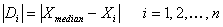All differences of zero are omitted.  Let the number of pairs remaining be denoted by n’, n’ < n.  Ranks from 1 to n’ are assigned to the n’ differences.  The smallest absolute difference |Di | is ranked 1, the second smallest |Di | is ranked 2, and so forth.  The largest absolute difference is ranked n’.  If groups of absolute differences are equal to each other, assign a rank to each equal to the average of the ranks they would have otherwise been assigned.  For example, if four absolute differences are equal and would hold ranks 8, 9, 10, and 11, each is assigned the rank of 9.5, which is the average of 8, 9, 10, and 11.

Although the absolute difference is used to obtain the rankings, the sign of Di is still used in the test statistic.  Ri, called the signed rank, is defined as follows:

Ri = the rank assigned to |Di | if Di  is positive.

Ri = the negative of the rank assigned to |Di | if Di  is negative.

The test statistic T+ is the sum of the positive signed ranks when there are no ties and n’ < 50.  Lower quantiles of the exact distribution of T+ are given in Table C-8. Under the null hypothesis that the Di s have mean 0.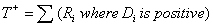Based on the relationship that the sum of the absolute differences is equal to n’ (n’ + 1) divided by 2, the upper quantiles wp are found by the relationship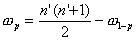If there are many ties, or if n’ > 50, the normal approximation test statistic T is used which uses all of the signed ranks, with their + and - signs.  Quantiles of the approximate distribution of T are given in a Normal Distribution Table.INTREPRETATION OF OUTPUT (DECISION RULE) OF WILCOXON SIGNED-RANKS TEST FOR SINGLE INDEPENDENT SAMPLE

For the two-sided test, reject the null hypothesis Ho at level a if T+ (or T) is less than its a/2 quantile or greater than its 1 - a/2 quantile from Table C-8 for T+ (or the normal distribution, see Table C-1 for T).  Otherwise, accept Ho (meaning the median (or mean) of X equals m).

For the upper-tailed test, reject the null hypothesis Ho at level a if T+ (or T) is greater than its a quantile from Table C-8 for T+ (or the Normal Table C-1 for T).  Otherwise, accept Ho (meaning the median (or mean) of X is less than or equal to m).  The p-value, approximated from the normal distribution, can be found byFor the lower-tailed test, reject the null hypothesis Ho at level a if T+ (or T) is less than its a quantile from Table C-8 for T+ (or the Normal Table C-1 for T).  Otherwise, accept Ho (meaning the median (or mean) of X is greater than or equal to m).  The p-value, approximated from the normal distribution, can be found byThe two-tailed p-value is twice the smaller of the one-tailed p-values calculated above.

Computational Example:  (Adapted from Conover (1999, p. 356-357)) Thirty observations on the random variable X are measured in order to test the hypothesis that E (X), the mean of X, is no smaller than 30 (lower-tailed test).

Ho:  E (X) (the mean) ³ m.

Ha: E (X) (the mean) < m.

The observations, the differences, Di  = (Xi - m), and the ranks of the absolute differences |Di | are listed below.  The thirty observations were ordered first for convenience.

Table 6: Ranking Statistics on 30 Observations of X

 Xi Di  = (Xi - 30) Rank of  |Di | Xi Di  = (Xi - 30) Rank of  |Di | 23.8 -6.2 17 35.9 +5.9 15* 26.0 -4.0 11 36.1 +6.1 16* 26.9 -3.1 8 36.4 +6.4 18* 27.4 -2.6 6 36.6 +6.6 19* 28.0 -2.0 5 37.2 +7.2 20* 30.3 +0.3* 1 37.3 +7.3 21* 30.7 +0.7* 2 37.9 +7.9 22* 31.2 +1.2* 3 38.2 +8.2 23* 31.3 +1.3* 4 39.6 +9.6 24* 32.8 +2.8* 7 40.6 +10.6 25* 33.2 +3.2* 9 41.1 +11.1 26* 33.9 +3.9* 10 42.3 +12.3 27* 34.3 +4.3* 12 42.8 +12.8 28* 34.9 +4.9* 13 44.0 +14.0 29* 35.0 +5.0* 14 45.8 +15.8 30*

There are no ties in the data nor is the sample size greater than 50.  Therefore, from Table C-8, Quantiles of Wilcoxon Signed Ranks Test Statistic, for n’ = 30, the 0.05 quantile is 152. The critical region of size £ 0.05 corresponds to values of the test statistic less than 152.  The test statistic T+ = 418.  This is the sum of all the Ranks, which have positive differences, as noted in the table by asterisks.  Since T+ is not within the critical region, Ho is accepted, and the analyst concludes that the mean of X is greater than 30.

The approximate p-value is calculated by the following equation.  Recall that the summation of the squares of a set of numbers from 1 to N is equal to [N (N+1) (2N + 1) / 6].The normal distribution table shows that the p-value is greater than 0.999 when the mean is no smaller than 30, i.e., there is a probability greater than 99.9% that the mean is greater than or equal to 30.

Mann-Whitney Test - Hypothesis About Population Means for Two Independent Samples

The Mann-Whitney test, sometimes referred to as the Mann-Whitney U test, is also called the Wilcoxon test.  There are actually two versions of the test that were independently developed by Mann and Whitney in 1947 and Wilcoxon in 1949.  They employ different equations and use different tables, but yield comparable results.  One typical situation for using this test is when the researcher wants to test if two samples have been drawn from different populations.  Another typical situation is when one sample was drawn, randomly divided into two sub-samples, and then each sub-sample receives a different treatment.

The Mann-Whitney test is often used instead of the t-test for two independent samples when the assumptions for the t-test may be violated, either the normality assumption or the homogeneity of variance assumption.  If a distribution function is not a normal distribution function, the probability theory is usually not available when the test statistic is based on actual data.  By contrast, the probability theory based on ranks, as used here, is relatively simple.  Additionally, according to Conover (1999), comparisons of the relative efficiency between the Mann-Whitney test and the two-sample t-test is never too bad while the reverse is not true. Thus the Mann-Whitney test is the safer test to use.

One can intuitively understand the statistics involved in this test.  First combine the two samples into a single sample and order them.  Then rank the combined sample without regard to which sample each value came from.  A test statistic could be the sum of the ranks assigned to one of the samples.  If the sum is too small or too great, this gives an indication that the values from its population tend to smaller or larger than the values from the other sample.  Therefore, the null hypothesis that there is no difference between the two populations can be rejected, if the ranks of one sample tend to be larger than the ranks of the other sample.

ASSUMPTIONS OF MANN-WHITNEY TEST FOR TWO INDEPENDENT SAMPLES

1)       Each sample is a random sample from the population it represents.

2)       The two samples are independent of each other.

3)       If there is a difference in the two population distribution functions F (x) and G (y), it is a difference in the location of the distributions.  In other words, if F (x) is not identical with G (y), then F (x) is identical with G (y + c), where c is some constant.

4)       The measurement scale is at least ordinal.

INPUTS FOR MANN-WHITNEY TEST FOR TWO INDEPENDENT SAMPLES

Let X1, X2, . . . , Xn represent a random sample of size n from population 1 and let Y1, Y2, . . . , Ym represent a random sample of size m from population 2.  Let n + m = N.  Assign ranks 1 to N to all the observations from smallest to largest, without regard from which population they came from.  Let R (Xi) and R (Yj) represent the ranks assigned to Xi and Yj for all i and j.  If several values are tied, assign each the average of the ranks that would have been assigned to them had there been no ties.

HYPOTHESES OF MANN-WHITNEY TEST FOR TWO INDEPENDENT SAMPLES

The Mann-Whitney test is unbiased and consistent when the four listed assumptions are met.  The inclusion of assumption 3 allows the hypotheses to be stated in terms of the means.  The expected value E (X) is the mean.

A.  Two-sided test

Ho:  E (X) = E (Y)

Ha:  E (X) ¹ E (Y)

B.  Upper-sided test

Ho:  E (X) ³ E (Y)

Ha:  E (X) < E (Y)

C. Lower-sided test

Ho:  E (X) £ E (Y)

Ha:  E (X) > E (Y)

The hypotheses shown here are for testing means.  Different hypotheses are also discussed in most texts (e.g., Conover (1999) and Sheskin (1997)) that test to see if the two samples come from identical distributions.  This does not require assumption 3.  Elsewhere in this chapter, the Kolmogorov-Smirnov type goodness-of-fit tests are described which also test if two (or more) samples are drawn from the same distribution.  For this reason, the identical distribution hypotheses of the Mann-Whitney test are not discussed here.

TEST STATISTIC FOR MANN-WHITNEY TEST FOR TWO INDEPENDENT SAMPLES

The test statistic T can be used when there are no ties or few ties.  It is simply the sum of the ranks assigned to the sample from population one.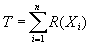If there are many ties, the test statistic T1 is obtained which simply subtracts the mean from T and divides by the standard deviation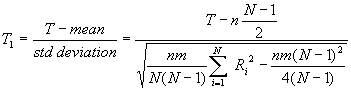where SRi2 is the sum of the squares of all N of the ranks or average ranks actually used in both samples.

Lower quantiles wp-1 of the exact distribution of T are given for n and m values of 20 or less in Table C-6. Upper quantiles wp are found by the relationshipPerhaps more convenient is the use T’ which can be used with the lower quartiles in Table C-6 whenever an upper-tail test is desired.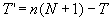When there are many ties in the data, T1 is used which is approximately a standard normal random variable.  Therefore, the quantiles for T1 are found in Table C-1, which is the standard normal table.

When n or m is greater than 20 (and there are still no ties), the approximate quantiles are found by the normal approximation given by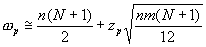for quantiles when n or m is greater than 20, where zp is the pth quantile of a standard normal random variable obtained from Table C-1.

INTREPRETATION OF OUTPUT (DECISION RULE) OF MANN-WHITNEY TEST FOR TWO INDEPENDENT SAMPLES

For the two-sided test, reject the null hypothesis Ho at level a if T (or T1) is less than its a/2 quantile or greater than its 1 - a/2 quantile from Table C-6 for T (or from the Standard Normal Table C-1for T1).  Otherwise, accept Ho if T (or T1) is between, or equal to one of, the quantiles indicating the means of the two samples are equal.

For the upper-tailed test, large values of T indicate that H1 is true.  Reject the null hypothesis Ho at level a if T (or T1) is greater than its a quantile from Table C-6 for T (or from the Standard Normal Table C-1 for T1).  It may be easier to find T’ = n (N+1) - T and reject Ho if T’ is less than its a from Table C-6. Otherwise, accept Ho if T (or T1) is less than or equal to its a quantile indicating the mean of population 1 is less than or equal to the mean of population 2.

For the lower-tailed test, small values of T indicate that H1 is true.  Reject the null hypothesis Ho at level a if T (or T1) is less than its a quantile from Table C-6 for T (or from the Standard Normal Table C-1 for T1).  Otherwise, accept Ho if T (or T1) is greater than or equal to its a quantile indicating the mean of population 1 is greater than or equal to the mean of population 2.  If the n or m is larger than 20, use

When n or m is greater than 20 (and no ties), the quantiles used in the above decisions are obtained directly from the equation given previously for this condition.

Computational Example:  (Adapted from Conover (1999, p. 278-279)) Nine pieces of flint were collected for a simple experiment, four from region A and five from region B.  Hardness was judged by rubbing two pieces of flint together and observing how each was damaged.  The one having the least damage was judged harder.  Using this method all nine pieces of flint were tested against each other, allowing them to be rank ordered from softest (rank 1) to hardest (rank 9).

Table 7: Hardness of Flint Samples from Regions A and B

 Region Rank A 1 A 2 A 3 B 4 A 5 B 6 B 7 B 8 B 9

The hypothesis to be tested is

Ho:  E (X) = E (Y) or the flints from regions A and B have the same means

Ha:  E (X) ¹ E (Y) or the flints do not have the same mean

The Mann-Whitney two-sided test is used with n = 4 and m = 5.  The test statistic T is calculated by

T = sum of the ranks of flints from region A

T = 1 + 2 + 3 + 5 = 11

The two-sided critical region of approximate size a = 0.05 corresponds to values of T less than 12 and greater than 28, which is calculated by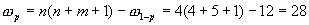Since the test statistic of 11 falls inside the lower critical region, less than 12, the null hypothesis Ho is rejected and the alternate hypothesis is accepted, i.e., the flints from the two regions have different harnesses.  Because the direction of the difference, it is further concluded that the flint in region A is softer than the flint in region B.

Safety Example:  In an evaluation of marked and unmarked crosswalks (Gibby, Stites, Thurgood, and Ferrara, Federal Highway Administration FHWACA/TO-94-1, 1994), researchers in California investigated if marked crosswalks at intersections had a higher accident frequency than unmarked crosswalks.  The data was analyzed in four major subsets: (1) all intersections, (2) intersections with accidents, (3) intersections with signals, and (4) intersections without signals.  Each of these was further dived into (1) intersections with crosswalks on the state highway approaches only and (2) intersections with crosswalks on all approaches.  This approach provided many subsets of data for analysis.

Two hypotheses were tested for each subset of data using a two-sided Mann-Whitney Test for two independent samples:

Ho: There is no difference between accident rates on marked and unmarked crosswalks.

Ha: There is a difference between the accident rates on marked and unmarked crosswalks.

A 5% or less level of significance was used as being statistically significant.

Several tests were made for each subset for which the researchers had sufficient data.  In some of the tests, the sample size was greater than 20 so the approximate quantiles were found by the normal approximation.  Where sample sizes were less than this, the quantiles were calculated according to the appropriate formula that matched the specific reference tables used by the researchers.  From these numerous tests, they were able to draw these general conclusions:

1. At unsignalized intersections marked crosswalks clearly featured higher pedestrian-vehicle accident rates.

2. At signalized intersections the results were inconclusive.

It should be noted that the names of many nonparametric test are not standardized.  In this study the researchers refer to the test they used as a Wilcoxon Rank Sum Test.  Their test is the same as that called the Mann-Whitney Test for Two Independent Samples in this manual.  Further, they used a different reference for their test than cited here.  This means they used a slightly different form of the test statistic than used in this manual, which corresponded to the tables in their reference versus the tables in the reference cited in this manual.  This example emphasizes the care that must be taken when applying nonparametric tests regarding matching the test statistic employed with its specific referenced tables.  One should not, for example, use a test statistic from this manual with tables from some other source.

Kruskal-Wallis One-Way Analysis Of Variance By Ranks Test For Several Independent Samples

Kruskal and Wallis (1952) extended the Mann-Whitney method to be applicable to two or more independent samples.  The typical situation is to test the null hypothesis that all medians of the populations represented by k random samples are identical against the alternative that at least two of the population medians are different.

The experimental design that is usually a precursor to applying this test is called the completely randomized design.  This design allocates the treatments to the experimental units purely on a chance basis.  The usual parametric method of analyzing such data is called a one-way analysis of variance or sometimes is referred to as a single-factor between-subjects analysis of variance.  This parametric method assumes normal distributions in using the F test analysis of variance on the data.  Where the normality assumption is unjustified, the Kruskal-Wallis test can be used.

ASSUMPTIONS OF KRUSKAL-WALLIS ONE-WAY ANALYSIS OF VARIANCE BY RANKS TEST FOR SEVERAL INDEPENDENT SAMPLES

1.       Each sample is a random sample from the population it represents.

2.       All of the samples are independent of each other.

3.       If there is a difference in any of the k population distribution functions F (x1), F (x2), ..., F (xk), it is a difference in the location of the distributions.  For example, if F (x1) is not identical with F (x2), then F (x1) is identical with F (x2 + c), where c is some constant.

4.       The measurement scale is at least ordinal.

INPUTS FOR KRUSKAL-WALLIS ONE-WAY ANALYSIS OF VARIANCE BY RANKS TEST FOR SEVERAL INDEPENDENT SAMPLES

The data consist of several random samples k of possibly different sizes.  Describe the ith sample of size ni by Xi1, Xi2, . . . , Xini,  The data can be arranged in k columns, each column containing a sample denoted by Xi,j where i = 1 to k and j = 1 to ni for each i sample.

Table 8: Input Table for Kruskal-Wallis One-Way Analysis of Variance

 sample 1 sample 2 . . . sample k X1,1 X2,1 . . . Xk,1 X1,2 X2,2 . . . Xk,2 . . . . . . . . . . . . X1,n1 X2,n2 . . . Xk,nk

The total number of all observations is denoted by N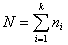total number of observations from all samples

Rank the observations Xij in ascending order and replace each observation by its rank R (Xi j), with the smallest observation having rank 1 and the largest observation having rank N.  Let Ri be the sum of the ranks assigned to the ith sample.  Compute Ri for each sample.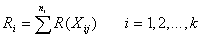If several values are tied, assign each the average of the ranks that would have been assigned to them had there been no ties.

HYPOTHESES OF KRUSKAL-WALLIS ONE-WAY ANALYSIS OF VARIANCE BY RANKS TEST FOR SEVERAL INDEPENDENT SAMPLES

Because the Kruskal-Wallis test is sensitive against differences among means, it is convenient to think of it as a test for equality of treatment means.  The expected value E (X) is the mean.

Ho:  E (X1) = E (X2) = . . . = E (Xk)   all of the k population means are equal

Ha:  At least one of the k population means is not equal to at least one of the other population means

The hypothesis shown here is for testing means as used in Montgomery (1997) as an alternative to the standard parametric analysis of variance test.  Sheskin (1997) and Conover (1999) state the null hypothesis in terms of all of the k population distribution functions being identical.  This difference has no practical effect in the application of this test.  The researcher is directed to the goodness-of-fit tests described elsewhere in this Chapter for tests regarding the equality of distribution functions.

TEST STATISTIC FOR KRUSKAL-WALLIS ONE-WAY ANALYSIS OF VARIANCE BY RANKS TEST FOR SEVERAL INDEPENDENT SAMPLES

When there are ties, the test statistic T is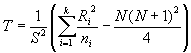S2 is the variance of the ranks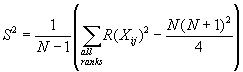If there are no ties, S2 = N (N + 1)/12 and the test statistic simplifies to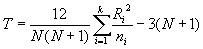When the number of ties is moderate, this simpler equation may be used with little difference in the result when compared to the more complex equation need for ties.

INTREPRETATION OF OUTPUT (DECISION RULE) OF KRUSKAL-WALLIS ONE-WAY ANALYSIS OF VARIANCE BY RANKS TEST FOR SEVERAL INDEPENDENT SAMPLES

The tables required for the exact distribution of T would be quite extensive considering that every combination of sample sizes for a given k would be needed, multiplied by however many samples k would be included.  Fortunately, if ni are reasonably large, say ni ³ 5, then under the null hypothesis T is distributed approximately as chi-square with k - 1 degrees of freedom, Ck-1.  For k =3, sample sizes less than or equal to 5, and no ties, consult tables in Conover (1999).

Reject the null hypothesis Ho at level a if T is greater than its 1 - a quantile.  This 1 - a quantile can be approximated by the chi-square Table C-2 with k-1 degrees of freedom.  Otherwise, accept Ho if T is less than or equal to the 1 - a quantile indicating the means of all the samples are equal in value.  The p-value is approximated by the probability of the chi-square random variable with k - 1 degrees of freedom exceeding the observed value of T.

Computational Example:  (Adapted from Montgomery (1997, p. 144-145)) A product engineer needs to investigate the tensile strength of a new synthetic fiber that will be used to make cloth for an application.  The engineer is experienced in this type of work and knows that strength is affected by the percent of cotton (by weight) used on the blend of materials for the new fiber.  He suspects that increasing the cotton content will increase the strength, at least initially.  For his application, past experience tells him that to have the desired characteristics the fiber must have a minimum of about 10% cotton but not more than about 40% cotton.

To conduct this experiment, the engineer decides to use a completely randomized design, often called a single-factor experiment.  The engineer decides on five levels of cotton weight percent (k samples) and to test five specimens (called replicates) at each level of cotton content (ni for each sample is 5) the fibers are made and tested in random order to prevent effects of unknown “nuisance” variables, e.g., if the testing machine calibration deteriorates slightly with each test.

The test results of all 25 observations are combined, ordered, and ranked.  Tied values are given the average of the ranks that they would have been assigned to them had there been no ties.  The test results and the ranks are shown in the following table.

Table 9: Kruskal-Wallis One-Way Analsis of Variance
by Ranks Test for Cotton Example

Weight Percent of Cotton15

20

25

30

35

X1j

R (X1j)

X2j

R (X2j)

X3j

R (X3j)

X4j

R (X4j)

X5j

R (X5j)

7

2.0

12

9.5

14

11

19

20.5

7

2.0

7

2.0

17

14

18

16.5

25

25

10

5

15

12.5

12

9.5

18

16.5

22

23

11

7.0

11

7.0

18

16.5

19

20.5

19

20.5

15

12.5

9

4

18

16.5

19

20.5

23

24

11

7.0

Ri =

27.5

66.0

85.0

113.0

33.5

ni =

5

5

5

5

5

N = 25

An example of how ties are ranked can be seen from the lowest value, which is 7.  Note that there are three observations that have a value of 7 so these would normally have ranks 1, 2, and 3.  Since they are tied, they are averaged and each value of 7 gets the rank of 2.0.

The hypothesis to be tested is

Ho:  E (X1) = E (X2) = . . . = E (Xk) all of the 5 blended fibers, with different percent weights of cotton, have mean tensile strengths that are equal

Ha:  At least one of the 5 blended fiber mean tensile strengths is not equal to at least one of the other blended fiber mean tensile strengths

Since there are ties, the variance of the ranks S2 is calculated by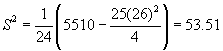The test statistic is calculated by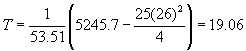For a critical region of 0.05, the 1 - a quantile (0.95) of the chi-square distribution with 5 - 1 = 4 degrees of freedom from Table C-2 is 9.49.  Since T = 19.06 lies in this critical region, i.e., in the region greater than 9.488, the null hypothesis Ho is rejected and it is concluded that at least one pair of the blended fiber tensile strength means is not equal to each other.

PAIRWISE COMPARISONS USING THE KRUSKAL-WALLIS ONE-WAY ANALYSIS OF VARIANCE BY RANKS TEST FOR SEVERAL INDEPENDENT SAMPLES

When the Kruskal-Wallis test rejects the null hypothesis, it indicates that one or more pairs of samples do not have the same means but it does not tell us which pairs.  Various sources support different methods for finding the specific pairs that are not equal, called pairwise comparisons.  Conover (1990) discusses using the usual parametric procedure, called “Fisher’s least significant difference,” computed on the ranks instead of the data.  If, and only if, the null hypothesis is rejected, the procedure dictates that the population means i and j seem to be different if this inequality is satisfied.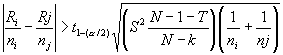Ri and Rj are the rank sums of the two samples being compared.  The 1 - a/2 quantile of the t distribution, t1-(a/1), with N - k degrees of freedom is obtained from the t distribution Table C-4.  S2 and T are as already defined for the Kruskal-Wallis test.

For the fiber tensile strength computational example, the pairwise comparisons between the 15% and the 20% cotton content fibers can be made by the following computation.  For a critical region of 0.05, from Table C-4, the 1 - a/2 quantile  (0.975) for the t distribution with 25 -5 = 20 degrees of freedom is 2.086.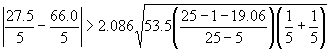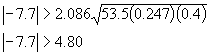Since the inequality is true, it is concluded that the tensile strength means of the 15% and the 20% cotton content fibers are different.  Notice that since all the samples are the same size, the right side of this equality will remain constant for all comparisons.  The following table lists all the pairwise comparisons.

Table 10: Pairwise Comparisons for Cotton Content Example

 Cotton Contents (% by wt)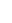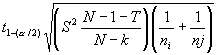15% and 20% 7.7 4.80 15% and 25% 11.5 4.80 15% and 30% 17.2 4.80 15% and 35% 1.2 4.80 20% and 25% 3.8 4.80 20% and 30% 9.4 4.80 20% and 35% 6.5 4.80 25% and 30% 5.6 4.80 25% and 35% 10.3 4.80 30% and 35% 15.9 4.80

All of the pairwise inequalities are true except for two, the 15% and 35% pair and the 20% and 25%.  Based on the engineers originally stated experience, he suspects that the 35% fiber may be losing strength which would account for the 15% and 35% pair having the same tensile strength.  The equal strengths of the 20% and 25% strengths appears to indicate that little benefit is gained in tensile strength by this raise the cotton content as compared to, say, the increase from 15% to 20% or from 25% to 30%.  Of course, more testing is probably prudent now that this preliminary information is known.

Wilcoxon Matched-Pairs Signed-Ranks Test For Two Dependent Samples

While this test is said to be for two dependent samples, it is actually a matched pair that is a single observation of a bivariate random variable.  It can be employed in “before” and “after” observations on each of several subjects, to see if the second random variable in the pair has the same mean as the first one.  To be employed more generally, the Wilcoxon Matched-Pairs Signed-Ranks test requires that each of n subjects (or n pairs of matched subjects) has two scores, each having been obtained under one of the two experimental conditions.  A difference score Di is computed for each subject by subtracting a subject’s score in condition 2 from his score in condition 1. Thus, this method reduces the matched pair to a single observation.  The hypothesis evaluated is whether or not the median of the difference scores equals zero.  If a significant difference occurs, it is likely the conditions represent different populations.

The Wilcoxon Matched-Pairs Signed Ranks Test was introduced earlier as a median (mean) test under the name Wilcoxon Signed-Ranks Test for a Single Independent Sample.  In that test, pairs were formed between a value in the sample and the sample median (mean).  Here, the test is extended to an experiment design involving two dependent samples.  Other than the forming of the pairs, the rest of the procedures remain the same.

ASSUMPTIONS OF WILCOXON MATCHED-PAIRS SIGNED-RANKS TEST FOR TWO DEPENDENT SAMPLES

1.       The sample of n subjects is a random sample from the population it represents.  Thus, the Dis are mutually independent.

2.       The Dis all have the same mean.

3.       The distribution of the Dis is symmetric.

4.       The measurement scale of the Dis is at least interval.

INPUTS FOR WILCOXON MATCHED-PAIRS SIGNED-RANKS TEST FOR TWO DEPENDENT SAMPLES

The data consist of n observations (x1, y1), (x2, y2), . . . , (xn, yn) on the respective bivariate random variables (X1, Y1), (X2, Y2), . . . , (Xn, Yn).

HYPOTHESES OF WILCOXON MATCHED-PAIRS SIGNED-RANKS TEST FOR TWO DEPENDENT SAMPLES

A.  Two-sided test

Ho:  E (D) = 0    or    E (Yi) = E (Xi)

Ha:  E (D) ¹ 0

B.  Upper-sided test

Ho:  E (D) £ 0    or    E (Yi) £ E (Xi)

Ha:  E (D) > 0

C. Lower-sided test

Ho:  E (D) ³ 0    or    E (Yi) ³ E (Xi)

Ha:  E (D) < 0

TEST STATISTIC |Di | OF WILCOXON MATCHED-PAIRS SIGNED-RANKS TEST FOR TWO DEPENDENT SAMPLES

The ranking is done on the absolute values of the differences between X and Y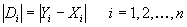All differences of zero are omitted.  Let the number of pairs remaining be denoted by n’, n’ < n.  Ranks from 1 to n’ are assigned to the n’ differences.  The smallest absolute difference |Di | is ranked 1, the second smallest |Di | is ranked 2, and so forth.  The largest absolute difference is ranked n’.  If groups of absolute differences are equal to each other, assign a rank to each equal to the average of the ranks they would have otherwise been assigned.

Although the absolute difference is used to obtain the rankings, the sign of Di  is still used in the test statistic.  Ri, called the signed rank, is defined as follows:

Ri = the rank assigned to |Di | if Di  is positive.

Ri = the negative of the rank assigned to |Di | if Di  is negative.

The test statistic T+ is the sum of the positive signed ranks when there are no ties and n’ < 50.  Lower quantiles of the exact distribution of T+ are given in Table C-8, under the null hypothesis that the Di s have mean 0.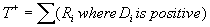Based on the relationship that the sum of the absolute differences is equal to n’ (n’ + 1) divided by 2, the upper quantiles wp are found by the relationship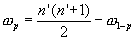If there are many ties, or if n’ > 50, the normal approximation test statistic T is used which uses all of the signed ranks, with their + and - signs.  Quantiles of the approximate distribution of T are given in a Normal Distribution Table.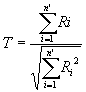INTREPRETATION OF OUTPUT (DECISION RULE) OF WILCOXON MATCHED-PAIRS SIGNED-RANKS TEST FOR TWO DEPENDENT SAMPLES

For the two-sided test, reject the null hypothesis Ho at level a if T+ (or T) is less than its a/2 quantile or greater than its 1 - a/2 quantile from Table C-8 for T+ (or the Normal Table C-1 for T).  Otherwise, accept Ho indicating the means of the two conditions are equal.

For the upper-tailed test, reject the null hypothesis Ho at level a if T+ (or T) is greater than its a quantile from Table C-8 for T+ (or the Normal Table C-1 for T).  Otherwise, accept Ho indicating the mean of Yi is less than or equal to the mean of Xi.

For the lower-tailed test, reject the null hypothesis Ho at level a if T+ (or T) is less than its a quantile from Table C-8 for T+ (or the Normal Table C-1 for T). Otherwise, accept Ho indicating the mean of Yi is greater than or equal to the mean of Xi.

Computational Example:  (Adapted from Conover (1999, p. 355)) Researchers wanted to compare identical twins to see if the first-born twin exhibits more aggressiveness than the second born twin does.  The Wilcoxon Matched-Pairs Signed-Ranks test is often used where two observations (two variables) are the matched-pairs for a single subject.  Here it is used where two subjects are the matched pairs for a single observation (variable).  So each pair of identical twins is the matched-pair and the measurement for aggressiveness is the single observation.

Twelve sets of identical twins were given psychological tests that were reduced to a single measure of aggressiveness. Higher scores in the following table indicate high levels of aggressiveness.  The sixth twin set has identical scores so they are removed from the ranking.

Table 11: Identical Twin Pyschological Test Example

 Twin Set 1 2 3 4 5 6 7 8 9 10 11 12 Firstborn Xi 86 71 77 68 91 72 77 91 70 71 88 87 Second born Yi 88 77 76 64 96 72 65 90 65 80 81 72 Difference Di +2 +6 -1 -4 5 0 -12 -1 -5 +9 -7 -15 Ranks of |Di| 3 7 1.5 4 5.5 na 10 1.5 5.5 9 8 11 Ri 3 7 -1.5 -4 5.5 na -10 -1.5 -5.5 9 -8 -11

The hypotheses to be tested are the lower-sided test

Ho:  E (D) ³ 0    or    E (Yi) ³ E (Xi) or E (Xi) £ E (Yi):  The firstborn twin (E (Xi)) does not tend to be more aggressive than the second born twin (E (Yi))

Ha:  E (D) < 0    or    E (Yi) < E (Xi) or E (Xi) > E (Yi):  The firstborn twin tends to be more aggressive than the second born twin.

There are several ties so the test statistic is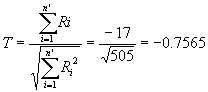For a critical region of size 0.05, the a quantile from the standard normal Table C-1 is -1.6449.  Since T = -0.7565 is not in this critical region, the null hypothesis Ho is accepted and it is concluded that the firstborn twin does not exhibit more aggressiveness than does the second born twin.

Traffic Example:  In a comparative study of modeling approaches for traffic flow forecasting (Smith and Demetsky, Journal of Transportation Engineering, V.123, N.4, July/August, 1997), researchers needed to assess the relative merits of four models they developed.  These traffic-forecasting models were developed and tested using data collected at two sites in Northern Virginia.  Two independent sets of data were collected for model development and model evaluation.  The models were estimated using four different techniques from the development data: historic average, time-series, neural network, and nonparametric regression (nearest neighbor).

One of the comparative measures used was the absolute error of the models.  This is simply how far the predicted volume deviates from the actual observed volume, using the model evaluation data.  The data were paired by using the absolute error experienced by two models at a given prediction time.  The Wilcoxon Matched-Pairs Signed-Ranks Test for dependent samples was used to assess the statistical difference in the absolute error between any two models.  This test was chosen over the more traditional analysis of variance approach (ANOVA) because the distribution of the absolute errors is not normal, an assumption required for ANOVA.

One of the models could not be tested because of insufficient data, leaving three models to be compared.  These models were compared using three tests, representing all combinations of comparison among three models.  Two hypotheses were tested for each pair of models:

Ho: m1 -m1 = 0

Ha: | m1 -m1 ¹ 0 | (note: the paper states the alternate hypothesis as m1 -m1 > 0, which is incorrect for a two-sided test, but its evaluation is correct      meaning that it actually evaluated as if it were a two-sided test.)

A 1% or less level of significance was used as being statistically significant.

Data from two sites were used, so the three tests were performed twice.  Although not stated specifically in the paper, it appears that the sample size was greater than 50.  This allowed the researchers to use the normal approximation test statistic.  For each of the two sites, the nonparametric regression (nearest neighbor) model was the preferred model.  Using this evidence, as well as other qualitative and logical evidence, the researchers were able to draw the conclusion that nonparametric regression (nearest neighbor) holds considerable promise for application to traffic flow forecasting.

A technique employed in this evaluation has universal application.  The test selected only compares two samples.  Therefore if more samples need to be compared, one can perform a series of tests using all the possible combinations for the number of samples to be evaluated.  It should be noted that often more sophisticated methods for testing multiple samples simultaneously usually exist.  Often these tests require more detailed planning prior to collecting the data.  Unfortunately many researchers collect data with only a vague notion of how the data will ultimately be analyzed.  This often limits the statistical methods available to them--usually to their detriment.  The next test in this manual, Friedman Two-Way Analysis of Variance by Ranks Test for several dependent variables, is an alternative that may have provided more sophisticated evaluation for these researchers, if their data had been drawn properly.

Friedman Two-Way Analysis Of Variance By Ranks Test For Several Dependent Variables

The situation arises where a matched-pair is too limiting because more than two treatments (or variables) need to be tested for differences.  Such situations occur in experiments that are designed as randomized complete block designs.  Recall that the Kruskal-Wallis One-Way Analysis of Variance by Ranks test was applied to a completely randomized design; this design, which relies solely on randomization, was used to cancel distortions of the results that might come from nuisance factors.  A nuisance factor is a variable that probably has an effect on the response variable, but is not of interest to the analyst.  It can be unknown and therefore uncontrolled, or it can be known and not controlled.  However, when the nuisance factor is known and controllable, then a design technique called blocking can be used to systematically eliminate its effects.  Blocking means that all the treatments are carried out on a single experimental unit.  If only two treatments were applied, then the experimental unit would contain the matched-pair treatments, which was the subject of the previous section on the Wilcoxon Matched-Pairs Signed-Ranks test.  This section discussed a test used when more than two treatments are applied (or more than two variables are measured).

The situation of several related samples arises in an experiment that is designed to detect differences in several possible treatments.  The observations are arranged in blocks, which are groups of experimental units similar to each other in some important respects.  All the treatments are administered once within each block.  In a typical manufacturing experiment, for example, each block might be a piece of material bi that needs to be treated with several competing methods xi.  Several identical pieces of material are manufactured, each being a separate block.  This would cause a problem if a completely randomized design were used because, if the pieces of material vary, it will contribute to the overall variability of the testing.  This can be overcome by testing each block with each of the treatment.  By doing this, the blocks of pieces of material form a more homogeneous experimental unit on which to compare the treatments.  This design strategy effectively improves accuracy of the comparisons among treatments by eliminating the variability of the blocks or pieces of materials.  This design is called a randomized complete block design.  The word “complete” indicates that each block contains all of the treatments.

The randomized complete block design is one of the most widely used experimental designs.  Units of test equipment or machinery are often different in their operating characteristics and would be a typical blocking factor.  Batches of raw materials, people, and time are common nuisance sources of variability in transportation experiments that can be systematically controlled through blocking.  For example, suppose you want to test the effectiveness of 4 sizes of lettering on signage.  You decide you will measure 10 people’s reactions and want a total sample size of 40.  This allows 10 replicates of each size of lettering.  If you simply assign the 40 tests (10 for size 1, 10 for size 2, 10 for size 3, and 10 for size 4) on a completely random basis to the 10 people, the variability of the people will contribute to the variability observed in the people’s reactions.  Therefore, each person can be blocked by testing all four lettering sizes on each person.  This will allow us to compare the lettering sizes without the high variability of the people confusing the results of the experiment.

An alternate test to the Quade test was developed much earlier (1937) by noted economist Milton Friedman.  The Friedman test is an extension of the sign test and is better known.  Which test to use depends on the number of treatments.  Conover (1999) recommends the use of the Quade test for three treatments, the Friedman test for six or more treatments, and either test for four or five treatments.  These recommendations are based on the power of the tests for various levels of treatments.  Since the Friedman test is useful for a much larger range of treatments, it is discussed in this section.  Discussion of the Quade test is presented in Conover (1999, p. 373-380).

ASSUMPTIONS OF FRIEDMAN TWO-WAY ANALYSIS OF VARIANCE BY RANKS TEST FOR SEVERAL DEPENDENT VARIABLES

1.       The multivariate random variables are mutually independent.  In other words, the results within one block do not affect the results within any of the other blocks.

2.       Within each block the observations may be ranked according to some criterion of interest.

INPUTS FOR FRIEDMAN TWO-WAY ANALYSIS OF VARIANCE BY RANKS TEST FOR SEVERAL DEPENDENT VARIABLES

The data consist of b mutually independent observations where each observation contains k random variables (Xi1, Xi2, . . . ,Xbk).  The observations are designated as b blocks, i = 1, 2, . . . , b.  The random variable Xij is in block i and is subjected to treatment j (or comes from population j).  The data can be arranged in a matrix with i rows for the blocks and j columns for the treatments (populations).

Table 12: Friedman Two-Way Analysis of Variance Table for Ranks Test
for Several Dependent Variables

 treatment 1 treatment 2 . . . treatment k block 1 X1,1 X1,2 . . . X1, k block 2 X2,1 X2,2 . . . X2, k . . . . . . . . . . . . . . . block b Xb,1 Xb,2 . . . Xb, k

HYPOTHESES OF FRIEDMAN TWO-WAY ANALYSIS OF VARIANCE BY RANKS TEST FOR SEVERAL DEPENDENT VARIABLES

Ho:  E (X1j) = E (X2j) = , . . . , = E (Xbj); All of the b treatment (population) means are equal, i.e., each ranking of the random variables within a block is equally likely.

Ha:  At least one of the b treatment (population) means is not equal to at least one of the other treatment (population) means

TEST STATISTIC FOR FRIEDMAN TWO-WAY ANALYSIS OF VARIANCE BY RANKS TEST FOR SEVERAL DEPENDENT VARIABLES

Current research reported by Conover (1999, p. 370) has found the approximation of the Friedman test statistic by the chi-square distribution is sometimes poor.  This supports a modification to the Friedman test statistic, which allows the F distribution to be used as its approximation with better results.

First rank the treatments (populations) within each block separately.  The smallest value within a block is assigned rank 1 continuing through the largest value, which is assigned rank k.  Use average ranks in case of ties.  Calculate the sum of the ranks Rj, for each treatment (population)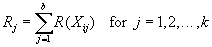Then calculate Friedman’s statistic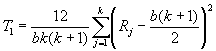When there are ties, T1 needs to be adjusted by A and C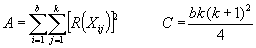After adjusting the test statistic T1 for ties, it becomes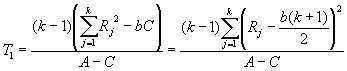Now the final test statistic T2 is calculated by modifying T1 so it can be approximated by the chi-square distribution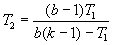INTREPRETATION OF OUTPUT (DECISION RULE) OF FRIEDMAN TWO-WAY ANALYSIS OF VARIANCE BY RANKS TEST FOR SEVERAL DEPENDENT VARIABLES

When the null hypothesis is true, the exact distribution of T2 is difficult to determine so its quantiles are approximated by the F distribution with numerator degrees of freedom dfnum = k - 1 and denominator degrees of freedom dfden = (b - 1)(k - 1).

Reject the null hypothesis Ho at level a if T2 is greater than its 1 - a quantile.  This 1 - a quantile s approximated by the F distribution Table C-5 with dfnum = k - 1 and dfden = (b - 1)(k - 1).  Otherwise, accept Ho if T2 is less than or equal to the 1 - a quantile indicating the means of all the samples are equal in value.  The approximate p-value can also be approximated from the F distribution table.  As one might suspect the approximation gets better as the number of blocks b gets larger.

Computational Example:  (Adapted from Montgomery (1997, p. 177-182)) A machine to test hardness can be used with four different types of tips.  The machine operates by pressing the tip into a metal specimen and the hardness is determined by the depth of the depression.  It is suspected that the four tip types do not produce identical readings so an experiment is devised to check this.  The researcher decides to obtain four observations (replicates) of each tip type.  A completely randomized single-factor design would consist of randomly assigning each of the 16 tests (called runs) to an experimental unit (a metal specimen).  This would require 16 different metal specimens.  The problem with this design is that if the 16 metal specimens differed in hardness, their variability would be added to any variability observed in the hardness data caused by the tips.

To overcome the potential variability in the metal specimens, blocking can be used to develop a randomized complete block design experiment.  The metal specimens will be the blocks.  Each block will be tested with all four tips.  Therefore, only four metal specimens will be needed to conduct the 16 total tests.  Within each block, the four tests need to be conducted in random order.  The observed response to the tests is the Rockwell C scale hardness minus 40 shown in the following table.

Table 13: Randomized Complete Block Design for Metal Specimens Example

 treatment (tip type) 1 treatment (tip type) 2 treatment (tip type) 3 treatment (tip type) 4 value rank value rank value rank value rank block specimen 1 9.3 2 9.4 3 9.2 1 9.7 4 block specimen) 2 9.4 2.5 9.3 1 9.4 2.5 9.6 4 block specimen 3 9.6 2 9.8 3 9.5 1 10.0 4 block specimen4 10.0 3 9.9 2 9.7 1 10.2 4 Rj (totals) 9.5 9 5.5 16

Since there are ties, first compute A and CNext compute the Friedman test statistic T1 using the formula adjusted for ties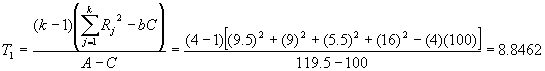Finally modify T1 to obtain the test statistic T2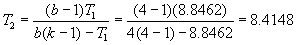For a critical region of 0.05, the 1 - a quantile (0.95) of the F distribution with dfnum = k - 1 = 3 and dfden = (b - 1)(k - 1) = (3)(3) = 9 from Table C-5 is 3.86.  Since T2 = 8.4148 lies in this critical region, i.e., in the region greater than 3.86, the null hypothesis Ho is rejected and it is concluded that at least one tip type results in hardness values that are not equal to at least one other tip type.  From Table C-5, the p-value is less than 0.01.  This means the null hypothesis Ho could have been rejected at a significance level as small as a = 0.01 (and even smaller, but most tables don’t have values any smaller).

MULTIPLE COMPARISONS USING FRIEDMAN TWO-WAY ANALYSIS OF VARIANCE BY RANKS TEST FOR SEVERAL DEPENDENT VARIABLES

When the Friedman test rejects the null hypothesis, it indicates that one or more treatments (populations) do not have the same means but it does not tell us which treatments.  One method to compare individual treatments is presented by Conover (1999, p. 371).  This method concludes that treatments l and m are different if the following inequality is satisfied.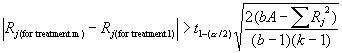Ri and Rj are the rank sums of the two treatments (samples) being compared.  The 1 - a/2 quantile of the t distribution, t1-(a/1), with (b - 1)(k - 1) degrees of freedom is obtained from the t distribution, Table C-4. A is as already defined for the Friedman test.

If there are no ties, A in the above inequality simplifies to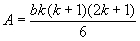Multiple comparisons for the hardness testing machine computational example can be made by first computing the right side of the inequality. For a critical region of 0.05, from Table C-4, the 1 - a/2 quantile  (0.975) for the t distribution with (4 - 1)(4 - 1) = 9 degrees of freedom is 2.262.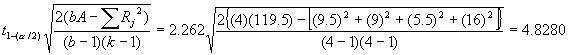It can be concluded that any two-tip types whose rank sums are more than 4.8280 are unequal.  Therefore, tip types which yield mean hardness values different from each other are types 1 and 4, types 2 and 4, and types 3 and 4.  No other differences are significant.  This means that types 1, 2, and 3 yield identical results (at a = 0.05) while type 4 yields a significantly higher reading than any of the other three tip types.

NONPARAMETRIC ANALYSIS OF BALANCED INCOMPLETE BLOCK DESIGNS

Sometimes it is inconvenient or impractical to administer all the treatments to each block.  Perhaps there is limited funds or perhaps the number of treatments is simply too large to administer to each block.  When blocking is used, but each block does not receive every treatment, it is called a randomized incomplete block design.  Furthermore, when certain simple treatment scheme conditions are met to aid analysis, the design is called a balanced incomplete block design.  The parametric analysis methods for this type of design are discussed in detail in Montgomery (1997, p. 208-219).  When the normality assumptions are not met, a test developed by Durbin in 1951 may be used.  It is a rank test to test the null hypothesis that there are no differences among treatments in a balanced incomplete block design.  For details about the Durbin test, see Conover (1999, p. 387-394).

Purposes of Measures of Association and Tests for Independence:

A measure of association describes the relationship between variables.  Another term often used is correlation, which is essentially synonymous with association.  Measures of association or correlation are descriptive statistical measures that attempt to quantify the degree of relationship between two or more variables.  After calculating a measure of association, it is usually possible to devise an inferential test to evaluate a hypothesis about the measure of association.  This section deals with nonparametric measures of association and tests for independence, i.e., measures and tests that do not rely on knowing the parameters of the underlying distributions of the variables being studied.  The focus is on comparing two variables, called a bivariate measure of association.

Examples: An analyst or engineer might be interested in assessing the evidence regarding the relationship (independent or not) between two variables, e.g., two variables such as:
1.   Airport site evaluation ordinal rankings of two sets of judges, i.e., citizens and airport professionals.
2.   Neighborhood districts residents’ use of a regional mall for purposes of planning transit routes.
3.   Accidents before and during roadway construction to investigate whether factors such as roadway grade, day of week, weather, etc. have an impact on crashes.4.   Area type and speed limit--as well as other variable pairs--to eliminate intercorrelated dependent variables for estimating models that predict the number of utility pole accidents.

Measures of Association and Tests for Independence Methodology: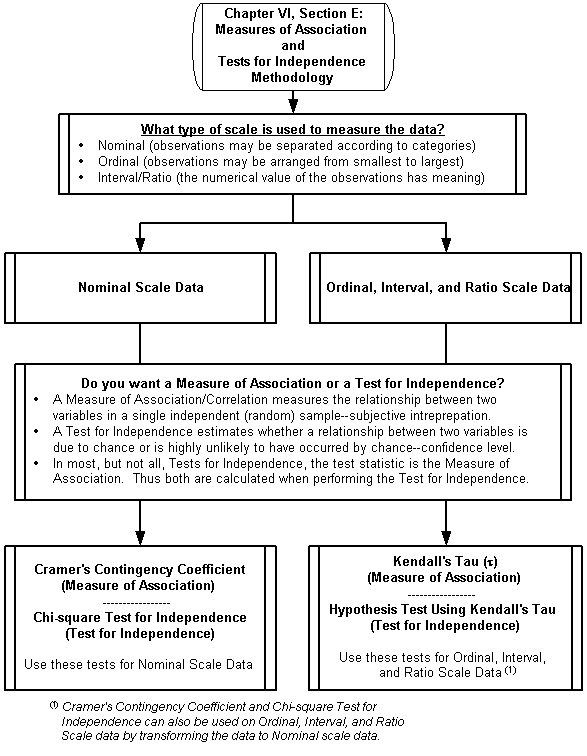Measures of Association and Tests for Independence Methodology:

What type of scale is used to measure the data?

As the reader will recall, there are four types of measurement scales that can be used to quantify data.  These are called nominal, ordinal, interval, and ratio scales, listed from “weakest” to “strongest.”  Thus the ratio scale is the strongest and gives us the most quantitative information of any of the scales while nominal gives us the least information.  This hierarchy allows for data to be transformed to a lower level, but they can’t be transformed to a higher one.  These measurement scales are defined in detail in Appendix A.

Do you want a Measure of Association or a Test for Independence?

Measures of Association:  The most commonly used measure of correlation is Pearson’s product-moment correlation coefficient, usually denoted by r. Pearson’s r was developed in 1896 and 1900 by Karl Pearson (1857-1936), an English mathematician.  Pearson was a pioneer of statistics who also developed the chi-square test that is used extensively in nonparametric methods.  Pearson’s r, however, is a random variable and therefore has a distribution function.  This distribution function depends on the bivariate distribution function of the two variables being tested.  Specifically, it assumes that each of the two variables and their linear combination are normally distributed--called a bivariate normal distribution.  Therefore, Pearson’s r is not useful as a test statistic in nonparametric methods.  Fortunately, other measures are available which do not assume a bivariate normal distribution of the two variables, which are detailed in this section.

In order for a quantitative measure of association to be useful, it must be scaled in a manner that implicitly yields information by its value.  Traditionally (but there are exceptions), the value calculated will fall between 0 and +1 or between -1 and +1.  A 0 value indicates no relationship between the two variables being compared.  A value of -1 or +1 indicates a maximum relationship between the two variables.  The closer to an absolute value of 1 (either +1 or -1), the stronger the measure of association.  When the measure can have a value from -1 to +1, the negative values indicate an inverse relationship (one increases as the other decreases) while the positive values indicate a positive relationship (both increase or decrease together).

It is important to remember that these relationships are only associations and do not imply causation.  One cannot say, based on a strong measure of association/correlation, that one variable causes another variable.  It is possible such a causal relationship exists but it cannot be discerned by using a measure of association/correlation.  Such a result could have been caused by an extraneous (often called a confounding) variable or variables that were not measured by the researcher.  These extraneous variables could be responsible for the observed correlation between the two variables.

Tests for Independence:  Measures of association/correlation are not inferential tests.  But as stated earlier, after calculating a measure of association, it is usually possible to devise an inferential test to evaluate a hypothesis about the measure of association.  As the reader will recall, hypothesis testing is the process of inferring from a sample whether or not a given statement about the population appears to be true.  The hypotheses discussed in this section can generally be employed in tests for independence.  This allows us to determine whether an association between two variables is due to chance or is highly unlikely to have occurred by chance.  Whereas a measure of association gives a quantitative measure of the relationship between two variables, its interpretation is subjective.  The interpretation of a test for independence allows the researcher to estimate a probability that the observed data occurs when the null hypothesis is true (the null hypothesis is usually that the variables are independent).  For example, there is less than a 5% probability that a specific data sample of two variables would occur given that the two data variables are independent.  In this example, since there is less than a 5% probability of the specific data occurring by chance alone, the given condition of independence could be rejected (at the 5% significance level).  This means that the alternative hypothesis would be accepted, i.e., the two variables are not independent and, thus, a relationship between them exists.

Measure of Association and Test for Independence for Nominal Scale Data

Cramer’s Contingency Coefficient - Measure of Association for Nominal Scale Data

The Test for Independence using nominal data discussed in the next section uses a r x c contingency table to explore whether two variables within a sample are independent or not.  But sometimes instead of testing a hypothesis regarding independence, the analyst simply want to express the degree of dependence shown in a particular contingency table.  The most widely used measure of dependence (also called a Measure of Association) for an r x c contingency table is Cramer’s Contingency Coefficient.  Sometimes called Cramer’s phi coefficient or simply Cramer’s coefficient, this measure of dependence was first suggested by Harold Cramer (1893-1985), a Swedish mathematician and chemist, in 1946.

ASSUMPTIONS OF CRAMER’S CONTINGENCY COEFFICIENT

The coefficient is based on the test statistic T developed in the Chi-square Test for Independence.  This test is detailed in the following section and all of its underlying assumptions apply to Cramer’s Contingency Coefficient.

INPUTS FOR CRAMER’S CONTINGENCY COEFFICIENT

A random sample of size N is obtained by the researcher.  The observations may be classified according to two variables.  The first variable has r categories (rows) and the second variable has c categories (columns).  Let Oij be the number of observations associated with row i and column j simultaneously (a cell).  The cell counts Oij are arranged in the following form, which is called a r x c contingency table.

The total number of observations from all samples is denoted by N.  The number of observations in the jth column is denoted by Cj, which is the number of observations that are in row j (meaning category j of the second variable). The number of observations in the ith row is denoted by Rj, which is the number of observations that are in row i (meaning category i of the first variable).

Table 14: Contingency Table for Two Variables

Column 1

Column 2

. . .

Column j

. . .

Column c

Row
Totals

Row 1

O1 1

O1 2

. . .

O1 j

. . .

O1 c

R1

Row 2

O2 1

O2 2

. . .

O2 j

. . .

O2 c

R2

. . .

. . .

Row i

O i 1

O i 2

. . .

O i j

. . .

O i c

Ri

. . .

. . .

Row r

O r 1

O r 2

. . .

O r j

. . .

O r c

RrColumn Totals

C1

C2

. . .

Cj

. . .

Cc

N

TEST STATISTIC (C) FOR CRAMER’S CONTINGENCY COEFFICIENT

The difficulty with directly using the test statistic T for the Chi-square Test for Independence is its lack of a common scale.  The test statistic T is an estimate of a chi-square value.  Chi-square values cannot be directly compared because their probabilities vary depending upon their degrees of freedom, which varies from sample to sample.  Cramer’s approach lessens this dependency on the degrees of freedom by dividing T by the maximum value that T can have.  The methodology for developing the test statistic T for the Chi-square Test for Independence is detailed in the following section and not repeated here.  However, it is easily seen by examining the test statistic T formula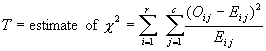that large values of T arise as the difference in cell counts (Oij - Eij) becomes more pronounced.  By examining extremely uneven contingency tables, a general rule was developed for the maximum value of T being N(k - 1), where k is the smaller of the number of categories for the two variables being considered and N is the total number of observations.  Therefore, when T is divided by the approximation of the maximum value of T, the result is a useful coefficient C having a common scale.  Current convention uses the square root form.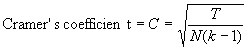INTREPRETATION OF OUTPUT OF CRAMER’S CONTINGENCY COEFFICIENT

If the result is close to 1.0, the contingency table indicates a strong dependency between the two variables; when it is close to 0.0, the numbers across each row (first variable) are in the same proportions to each other as the column totals (second variable) are to each other--indicating independence.

It should be noted that while measures of association have a quantitative scale, their interpretation is subjective.  The interpretation can be improved by performing the Test for Independence on the contingency table as detailed in the following section.  This test determines if the test statistic T (estimate of chi-square value) is statistically significant at a given level of significance.  The Measure of Association computed for the contingency table, in this case Cramer’s Contingency Coefficient will be significant at this same level of significance.  Finally, it is important to note once more that these relationships are only associations and do not imply causation.

Other Nonparametric Measures of Association for Nominal Scale Data

Whereas Cramer’s Contingency Coefficient is the most widely used measure of dependency for r x c contingency tables, other measures are sometimes used.  One widely know measure is Pearson’s Coefficient of Mean Square Contingency sometimes simply called Pearson’s Contingency Coefficient.  Detailed information on this measure and others is available from Conover (1999, p231-233) or Sheskin (1997, p.243-244).  The choice of a measure of dependency is a personal choice.

Chi-square Test for Independence (also called Cross Classification or Cross Tabulation) - Test for Independence for Nominal Scale Data

ASSUMPTIONS OF CHI-SQUARE TEST FOR INDEPENDENCE

1)       The sample of N observations is a random sample.

2)       Each observation may be categorized into exactly one of the r categories for one variable and c categories for the other variable (i.e., the r categories are mutually exclusive and collectively exhaustive as are the c categories)

3)       The number of observations that fall into each r category for one variable or for each c category for the other variable are not predetermined by the researcher prior to the data collection phase of a study.

4)       Each cell contains at least 5 observations (see the discussion under “Trouble shooting” about possible remedies if this assumption is violated).

Safety Example:  In a reexamination of a study to determine whether to use red left turn arrows instead of red balls (Kullback and Keegel, Journal of Transportation Engineering, V.111, N.4, July, 1985), researchers challenged the conclusions of the previous study because of inappropriate use of the Chi-square Test for Independence.  The original study had used a contingency table with 72 cells.  However 30 of these cells had zero observations, making the use of this test highly problematic.

5)       The measurement scale is at least nominal.

INPUTS FOR CHI-SQUARE TEST FOR INDEPENDENCE

The data consist of an r x c contingency table, as prepared for Cramer’s Contingency Coefficient detailed in the previous section.

HYPOTHESES OF CHI-SQUARE TEST FOR INDEPENDENCE

Let the probability of a randomly selected value from the ith population being classified in the jth class be denoted by pi j, for i = 1, 2, . . . , r, and  j = 1, 2, . . . , c.

Ho:  The two variables are independent of each other, i.e., the rows and columns represent two independent classification schemes.  (Oi j  = Ei j, for all cells).

Ha:  The two variables are not independent of each other. (Oi j  ¹ Ei j, for all cells).

TEST STATISTIC (T ) OF CHI-SQUARE TEST FOR INDEPENDENCE

The null hypothesis can be stated as the event “an observation is in row i” is independent of the event “that same observation is in column j,” for all i and j.  By definition, when two events are independent, the probability of both occurring is simply the product of their probabilities.  Thus, for any cell, assuming the null hypothesis is true (the variables are independent), the expected number of observations is the product of their category probabilities.  The row probability is simply the row total divided by all the observations, P (row i) = Ri /N.  Similarly, the column probability is the column total divided by all the observations, P (column j) = Cj /N.  Therefore the expected number of observations in a cell is the cell probability times the total number of observations in all the cellsWhere Ei j represents the expected number of observations in cell (i, j) when Ho is true.  The test statistic T is given by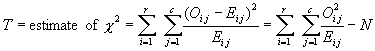INTEPRETATION OF OUTPUT (DECISION RULE) OF CHI-SQUARE TEST FOR INDEPENDENCE

The approximate distribution of T is the chi-square distribution with (r - )(c - 1) degrees of freedom. Therefore the critical region of approximate size a significance level corresponds to values of T greater than x1- a, the (1 - a) quantile of a chi-square random variable with (r - 1)(c - 1) degrees of freedom, obtained from the Chi-square distribution, Table C-2.  One rejects Ho if T exceeds x1-a (meaning that an observation’s categorization on the first variable is associated (i.e., not independent) with the categorization on the second variable).  Other wise the analyst accepts Ho (meaning the two variables are independent).

Transit Example:  In the design of off-peak transit routes (Ross and Wilson, Transportation Engineering Journal, V.103, N.TE5, September 1977), researchers in Cedar Rapids, Iowa, developed a routing technique to identify the trade area of major trip attractors and design the transit routing to serve areas of high potential within the trade area.  To demonstrate the effectiveness of the technique, the researchers collected data for a non-CBD (central business district) regional mall and its trade area.  The data captured demographic characteristics of the mall’s patrons and characteristics of their trips to the mall during off-peak hours.  Screening methods were used to eliminate from evaluation traffic zones of very low potential transit ridership to the mall, essentially all areas outside a 10-minute travel time to the mall.  Additionally, low population traffic zones within the 10-minute limit were also dropped, typically rural zones.  The remaining traffic zones were aggregated into four districts conducive to efficient transit routing to the mall.

Several questions were posed in the form of two hypotheses and tested using the Chi-square Test for Independence for nominal data:

Ho: The two variables are independent of each other.

Ha: The two variables are not independent of each other.

A 1% or less level of significance was used as being statistically significant and the p-values were reported when the level of significance was greater than 1%.

One example of a question explored was the preference of district residents for shopping at the mall when compared to the CBD.  The responses to this choice question were that they shopped (1) more, (2) the same, or (3) less in the CBD than in the mall.  Since there were four districts being evaluated, the researchers set up a 3 by 4 contingency table.  The null hypothesis is that the rows (level of shopping at the mall) are independent of the columns (the district in which the shopper lived).  This was rejected at a degree of significance of 0.01.  This is interpreted as follows. Given that the data are independent, there is less than a 1% chance that the large differences between the expected and observed values occurred by chance alone.  Therefore the alternate hypothesis is accepted: the level of shopping is different among the districts.  Several other questions were explored in a similar manner: (1) are the trip frequencies the same by district (no), (2) are the planned versus unplanned trips to the mall the same by districts (yes with a p-value = 0.58), (3) are the previous locations of the mall patrons just before coming to the mall the same by district (yes with a p-value of 0.70), (4) are the trip making rates per occupied dwelling unit the same by district (yes with a p-value > 0.70), and then (5) for each zone within a district, are the trip making rates per occupied dwelling unit the same (results varied by zone).

From these tests, the researchers were able to draw these general conclusions:

1.  After dropping the lowest use district, all three remaining districts appear to be producing trips to the mall as a function of the number of occupied dwelling units in the districts.

2.  In two of the districts at least one zone produces trips at a rate different than would be expected if all trip rates were equal.

APPROXIMATE VERSUS EXACT INFERENCES FOR CHI-SQUARE TEST FOR INDEPENDENCE AND CHI-SQUARE GOODNESS OF FIT TEST

Nonparametric techniques generate p-values without making any assumptions about the distributions.  However, they do rely on approximate solutions.  Recall the noted nonparametric authority W.J. Conover’s words: “nonparametric methods use approximate solutions to exact problems, while parametric methods use exact solutions to approximate problems.”  These approximations tend to become better as the sample size gets larger because it usually approaches its solution asymptotically or as some say, the law of large numbers comes into play.  So when samples sizes are sufficiently large the approximations are usually quite good.

For both the Chi-square Test for Independence and the Chi-square GOF test, the exact distribution of the test statistic is very difficult to find and classically is almost never used.  The asymptotic chi-square approximation for the test statistic is satisfactory if the expected values in the test statistic are not too small.  However the exact distribution can be found, and has been used for some time for small contingency tables, e.g., a 2 by 2 contingency table, where the computations required were manageable.  The computations for larger size contingency table are difficult computationally.  With the advent of modern computers, however, such computations are possible and may provide a more useful method when testing small size samples.  These computations are still a nontrivial task and rely on special algorithms.  Cyrus R. Mehta and Nitin R. Patel are two researchers who have developed such special algorithms and have published their work in this field.  They report that software support for their methods is available in many standard packages including StatXact-3, LogXact-2, SPSS Exact Tests, and SAS Version 6.11.  More statistical software publishers will probably add this capability as it becomes more recognized.  One should consult these software providers for more information.

Measure of Association and Test for Independence for Ordinal, Interval, and Ratio Scale Data

Kendall’s Tau (t) - Measure of Association for Ordinal, Interval, and Ratio Scale Data

Kendall’s Tau is of a type that can be called measures of rank correlation.  Compared to measures of association for nominal scale data, these measures use the additional information contained in ordinal, interval, and ratio scale data in their computation.  In general, this additional information provides tests with greater power without having to make the assumptions about the distribution of the test statistics as is the case for parametric tests (e.g., the widely used Pearson’s product moment correlation coefficient).  Rank correlation methods use the ranks (order) attributed to the data values rather than the values themselves resulting in nonparametric tests.

ASSUMPTIONS OF KENDALL’S TAU (t)

1)       P (X = x) < 1 which is a trivial assumption since it is unlikely the parent population that the sample is drawn from will have only one member (number).  This assumption, however, allows this test to be valid for all types of populations, whether continuous, discrete, or mixtures of the two.

2)       The measurement scale is at least ordinal.

INPUTS FOR KENDALL’S TAU (t)

The data consist of a random sample of size n having two variables (X, Y).

TEST STATISTIC (t) FOR KENDALL’S TAU

Slightly different versions of Kendall’s Tau are used by different researchers that require different tables. Described here is the method used by Conover (1990, p.319-323, TableA11 p.543).  Two observations, for example (10.4, 0.6) and (13.9, 1.1), are called “concordant” if both members (variables) of one observation are larger than their respective members of the other observation.  Let Nc be the number of concordant pairs of observations out of the nC2 possible pairs. A pair of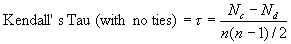observations, such as (10.4, 0.6) and (14.2, 0.3), are called “discordant” if the two numbers in one observation differ in opposite directions (one negative and one positive) from the respective members in the other observation.  Let Nd be the total number of discordant pairs of observations.  Pairs with ties between respective members are counted differently, as described later. Recall nCr = (n!) / (r! (n - r)!) are all the number of ways in which r objects can be selected from a set of n distinct objects.  Therefore, the n observations may be paired nC2 = (n!) / (2! (n - 2)!) = n (n - 1) / 2 different ways.  Thus, the number of concordant pairs Nc plus the number of discordant pairs Nd plus the number of pairs with dies will add up to n (n - 1) / 2.  The measure of correlation (association) when there are not ties is

If all pairs are concordant, then Kendall’s Tau equals 1.0; when all pairs are discordant, it equals ‑1.0.

Ties:  In equation form, a pair of observations (X1, Y1) and  (X2, Y2) is concordant if (Y2 - Y1) / (X2 - X1) > 0 and discordant if (Y2 - Y1) / (X2 - X1) < 0.  If X1 = X2 the denominator is zero so no comparison can be made.  But when Y1 = Y2 (and X1 ¹ X2), then (Y2 - Y1) / (X2 - X1) = 0.  In this case the pair should be counted as one-half (1/2) concordant and one-half (1/2) discordant.  While this makes no difference in the numerator of Kendall’s Tau because the one-half terms cancel when computing Nc - Nd, it does change the way Tau should be computed.  In the case of ties, the measure of association (correlation) is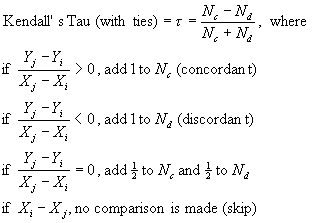This version of Kendall’s Tau has the advantage of achieving +1 or -1 even if ties are present.  This version is sometimes called the “gamma coefficient.”

INTREPRETATION OF OUTPUT OF KENDALL’S TAU (t)

If the result is close to 1.0, the data indicate a strong positive association/correlation.  This means the larger values of X tend to be paired with the larger values of Y and the smaller values of X tend to be paired with the smaller values of Y.  If the result is close to -1.0, the data indicate a strong negative association/correlation.  This means the larger values of X tend to be paired with the smaller values of Y and the smaller values of X tend to be paired with the larger values of Y.  A result close to 0.0 indicates the values of X seem to be randomly paired with the values of Y, and hence indicate that X and Y are independent.  However, independence has not been statistically tested so the most the analyst can say is that the variables are uncorrelated.

To statistically test the association (or lack of), the Test of Independence in the next section needs to be made.  Once that is made, however, and assuming the test is statistically significant at a given level of significance, then Kendall’s Tau will be significant at this same level of significance.  As with all Measures of Association, Kendall’s Tau only measures an association between two variables and do not imply that one causes the other.

Computational Example:  (Adapted from Conover (1999, p.320)) Suppose the analyst collected twelve observations, each having two variables for each observation.  The analyst wants to determine the Measure of Association using Kendall’s Tau between two variables. One of the variables is denoted as X (it doesn’t matter which one), and the other variable Y.  For ease of calculation, the observations are ordered on their X variable and calculate their concordant and discordant pairs as shown in the following table. Included are the ranks of the variables, which some people find easier to use when comparing.  You can use the values directly or their ranks to make the comparisons.  Ranking of ties uses their average rank.

By arranging the data in increasing values of X, each Y may be compared with only those Ys below it.  In this manner each pair is only considered once and the comparisons can be done by hand fairly quickly.  Using the pair (560, 3.2) with ranks (5, 1.5) as an example, the pair relationships are found by comparing 3.2 (or rank 1.5) with the following Ys of 3.2, 3.8, 3.5, 4.0, 3.9, and 4.0 (or with ranks 1.5, 9, 5, 11.5, 10, and 11.5).  In 5 comparisons the second Y is larger (concordant), in no cases is the second Y smaller (discordant), and in one case there is a tie (1/2 concordant and 1/2 discordant).  Note that the two pairs where 560 is tied with the second X are not counted.

Table 15: Kendall’s Tau Measure of Association between Two Varaiables

 Values (Xi,Yi) Ranks (Ri,Ri) Concordant Pairs Below (Xi,Yi) and (Ri,Ri) Discordant Pairs Below (Xi,Yi) and (Ri,Ri) (530, 3.5(1)) ( 1, 5) 7 4 (540, 3.3) ( 2, 3) 8 2 (545, 3.7) ( 3, 8) 4 5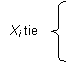(560, 3.2(1)) ( 5, 1.5) 5.5 0.5 (560, 3.5) ( 5, 5) 4.5 1.5 (560, 3.6) ( 5, 7) 4 2 (570, 3.2(1)) ( 7, 1.5) 5 0 (580. 3.8) (8, 9) 3 1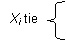(610, 3.5) (9.5, 5) 2 0 (610, 4.0(2)) (9.5, 11.5) 0.5 1.5 (640, 3.9) (11, 10) 1 0 (710, 4.0(2)) (12,.11.5 ) n/a n/a Nc = 44.5 Nd = 17.5

(1)Yi tie

(2)Yi tie

Safety Example:  In an evaluation of vehicular crashes with utility poles (Zegeer and Parker, Transportation Research Record No. 970, 1984), researchers used data from four states to investigate the effects of various traffic and roadway variables on the frequency and severity of the crashes.  Several methods were used to assess these effects including correlation analysis, analysis of variance and covariance, and contingency table analysis.

Correlation analysis was conducted to determine if a relationship existed between the independent and the dependent variables for purposes of determining the best variables to use in a predictive model.  Similarly correlation analysis between independent variables was conducted in order to avoid problems of collinearity in predictive models that occurs when two or more independent variables in a model are highly correlated.  For variables having interval and ratio scale data, the “Pearson correlation coefficient” was used.  For measuring the association between the discrete, ordinal independent variables, “Kendall Tau correlation” was used.  For example, a Tau value of 0.727 was reported for the correlation between area type and speed limit.  There were three area types: urban, urban fringe, and rural.  A value of 1.000 would indicate “perfect” correlation between these two variables while a value of 0.000 would indicates “no” correlation.  The researchers made a qualitative decision that this Tau value was sufficiently close to 1.0 to warrant eliminating one of the variables--area type--as an independent variable in their predictive models.  After deciding on the “best” variables to include, the researchers used linear and nonlinear regression analysis to develop predictive models.

It is important to note that when reporting results, lack of specificity in naming and/or describing the actual statistical tests used can cause confusion.  In this example, the researchers reported they used the “Pearson correlation coefficient”, except for ordinal data, for which they used “Kendall Tau correlation” analysis.  In neither case is a statistical reference given, which would have allowed a reader so inclined to determine the exact statistical tests that were used.  Both Pearson and Kendall formulated multiple tests for correlation and they are reported in literature and textbooks under various names.  In this case, “Kendall’s tau” is a relatively unique name but the Pearson correlation coefficient used must simply be guessed.  It is probably Pearson’s Product Moment Correlation Coefficient, which is arguably the most widely used correlation coefficient for interval and ratio scale variables when developing linear regression models.  An alternate method for providing specificity would be to include sample calculations or other descriptors of the results such that the specific test could be deduced by the reader.  Again, this is lacking in the example reported here.

Other Nonparametric Measures of Association for Ordinal, Interval, and Ratio Scale Data

Spearman’s Rho (r) is another measure of association that is historically more commonly discussed in statistical textbooks.  Its computation is a natural extension of the most popular parametric measure of association, Pearson’s product-moment correlation coefficient (r) mentioned earlier.  Spearman’s Rho is simply Pearson’s product-moment correlation coefficient computed using the ranks of the two variables instead of their values.  An advantage of Spearman’s Rho over Kendall’s Tau is that it is easier to compute, but this becomes moot when using many of today’s computer software programs which will compute both.

The primary advantage of Kendall’s Tau is that the sampling distribution of tau approaches normality very quickly.  Therefore, when the null hypothesis of independence is true, the normal distribution provides a good approximation of the exact sampling distribution of tau of small sample sizes--better that for Spearman’s Rho which requires a larger sample size for this approximation.  Another commonly cited advantage is that Kendall’s Tau is an unbiased estimate (i.e., the most accurate) of the population parameter tau whereas Spearman’s Rho is not an unbiased estimate (i.e., not as accurate) of the population parameter rho.

While Kendall’s Tau arguably provides a better measure of association that does Spearman’s Rho, it does not preclude the use of Spearman’s Rho.  On the contrary, Spearman’s Rho provides a useful nonparametric and should not be avoided, especially if, for example, it is the only one available in the researcher’s statistical software.  When hypothesis testing is used for Tests of Independence, both Kendall’s Tau and Spearman’s Rho will produce nearly identical results.

Hypothesis Test Using Kendall’s Tau (t)  - Test for Independence for Ordinal, Interval, and Ratio Scale Data

ASSUMPTIONS OF HYPOTHESIS TEST USING KENDALL’S TAU (t)

The coefficient is based on the test statistic Tau (t) developed in the Kendall’s Tau Measure of Association (t).  This test is detailed in a previous section and all of its underlying assumptions apply to this Hypothesis Test.

INPUTS FOR HYPOTHESIS TEST USING KENDALL’S TAU (t)

The data consist of a random sample of size n having two variables (X, Y) as detailed in a previous section for Kendall’s Tau as a measure of association.

HYPOTHESES OF THE HYPOTHESIS TEST USING KENDALL’S TAU (t)

Kendall’s Tau (t) can be used as the test statistic to test the null hypothesis of independence between the two variables (X, Y), with possible one or two tailed alternatives as described below.  The test involves determining if our computed value of Kendall’s Tau (t) is large enough or small enough to allow us to conclude that the underlying population correlation coefficient between the two variables is some value other than zero.

A.  Two-sided test

Ho:  The two variables are independent of each other, i.e., the two variables have zero correlation.

Ha:  The correlation between the two variables equals some value other than zero, i.e., pairs of observations either tend to be concordant or tend to be discordant.

B.  Upper-sided test

Ho:  The two variables are independent of each other, i.e., the two variables have zero correlation.

Ha:  The correlation between the two variables equals some value greater than zero, i.e., pairs of observations either tend to be concordant.

This is used when the variables are suspected of being positively correlated.

C. Lower-sided test

Ho:  The two variables are independent of each other, i.e., the two variables have zero correlation.

Ha:  The correlation between the two variables equals some value less than zero, i.e., pairs of observations tend to be discordant.

This is the one-side test to use when the variables are suspected of negatively correlated.

TEST STATISTIC (t) FOR HYPOTHESIS TEST USING KENDALL’S TAU

The methodology for developing the test statistic Kendall’s Tau (t) is detailed in a previous section and not repeated here.  The Tau (with no ties) should be used when there are no ties or few ties (a few can be tolerated with acceptable results).  If there are extensive ties then the Tau (with ties) should be used as the test statistic.

INTREPRETATION OF OUTPUT (DECISION RULE) OF HYPOTHESIS TEST USING KENDALL’S TAU (t)

For the two-sided test, reject the null hypothesis Ho at the level of significance a (meaning the two variables are correlated) if the test statistic Tau is less than the a/2 quantile or greater than its 1 - a/2 quantile in the null distribution tabulated in Table C-9, otherwise accept Ho (meaning the two variables are independent).

For the upper-sided test, reject the null hypothesis Ho at the level of significance a (meaning the two variables are positively correlated) if the test statistic Tau is greater than the 1 - a/2 quantile in the null distribution tabulated in Table C-9, otherwise accept Ho (meaning the two variables are independent). Similarly, for the lower-sided test, reject the null hypothesis Ho at the level of significance a (meaning the two variables are negatively correlated) if the test statistic Tau is less than the a/2 quantile in the null distribution tabulated in Table C-8, otherwise accept Ho (meaning the two variables are independent).

Purposes of Goodness of Fit Methods:

Goodness-of-fit methods infer how well a particular set of data fits a given distribution as a whole.  Such tests are designed to compare the sample obtained with the type of sample one would expect from the hypothesized distribution to see if the hypothesized distribution function “fits” the data in the sample.

Many of statistical inference methods detailed in this manual involve hypothesis testing.  Hypothesis testing is the process of inferring from a sample whether or not to accept a certain statement about the population.  Recall that a sample is a group of items (data points) selected from a parent population (all data points of which our sample is a subset) so that properties or parameters of the parent population (simply called population) may be estimated.  The statement under scrutiny is called the hypothesis.

Often the hypotheses being tested are statements about the unknown probability distribution of the data points being observed.  Examples include “The median is 4.0” and “The probability of being in category A is the same for both populations.”  These look only at parts of the unknown probability distribution, such as the median or at an isolated statement about some of the probabilities.  But it is often important to characterize the distribution as a whole.  In order to do this, statements must be tested about all of the parts simultaneously.  Two examples of these types of hypotheses are “The unknown distribution function is the normal distribution function with a mean of 2.5 and a variance of 1.75” and “The distribution function of this parent population is binomial, with parameters n = 10 and p - 0.2.”  These more comprehensive hypotheses may be tested with a goodness-of-fit test, the subject of this section.

The goodness-of-fit methods are not restricted to testing a single sample against a known distribution.  They can also be used to test if two (or more) samples are both drawn from the same, but unknown, distribution.  These samples are independent, i.e., drawing one sample from the population does not in any way affect the drawing of another sample from the population.  Although not discussed in this manual, the reader should be aware that goodness-of-fit tests are also available for dependent samples.  The reader is referred to the references listed at the beginning of this Chapter for more information on testing two or more dependent samples.

Examples: An analyst or engineer might be interested to assess the evidence regarding relative goodness of fit of:
1.   Candidate predictive models to the observed data for expected accident rates for rail-highway crossings.
2.   Air quality data to hypothetical probability distributions--lognormal, gamma, beta, Weibull and Johnson--with the intent of using the distributions to predict the number of days with observed ozone and carbon monoxide concentrations exceed National Ambient Air Quality Standards.

Goodness-of-Fit Methodology: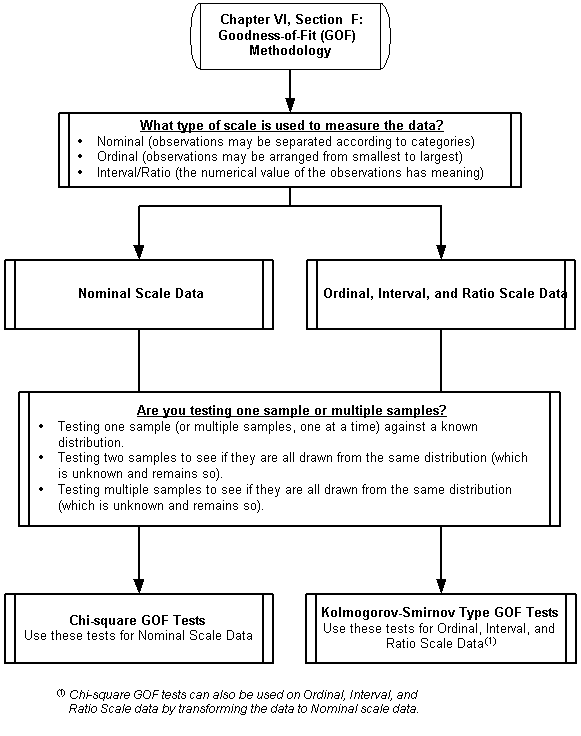Goodness-of-Fit (GOF) Methodology:

What As the reader will recall, there are four types of measurement scales that can be used to quantify data.  These are called nominal, ordinal, interval, and ratio scales, listed from “weakest” to “strongest.”  Thus the ratio scale is the strongest and gives us the most quantitative information of any of the scales while nominal gives us the least information.  This hierarchy allows for data to be transformed to a lower level, but they can’t be transformed to a higher one.  These measurement scales are defined in detail in Appendix A.

Are you testing one sample or multiple samples?

The type of test to be used depends on what the researcher is trying to determine about the distribution from which the data sample(s) is drawn.  Even for the same type of data scale, the following three possibilities lead to different test methods, or in some cases, variations of the same test method.

1.       Testing one independent sample (or multiple samples, tested one at a time) against a known distribution that is postulated by the researcher.  Usually the researcher has some clues as to what the postulated distribution might be.  This may come from the researcher’s prior experience or from that of another, perhaps gained through a literature search.  But regardless of how selected, the knowledge about the postulated distribution will have to be sufficient to provide the detailed comparison statistics needed by the GOF test.

2.       Testing two samples to see if they are drawn from the same distribution, which is unknown.  Sometimes the researcher does not want to find out which distribution a sample comes from but wants to know if two samples both come from the same distribution.  This is useful knowledge in a number of situations.  For example, a researcher may have two instruments for sampling data; one instrument is believed to sample the data more accurately but costs considerably more in time and money than the other method.  The researcher can draw samples with each instrument and test to see if they are drawn from the same underlying distribution.  If so, then the researcher is beginning to build a case for using the cheaper instrument as being adequate to sample the data, even though it is less accurate.

3.       Testing multiple samples to see if they are all drawn from the same distribution, which is unknown and remains so.  This is an extension of the two-sample case, but here the researcher wants to simultaneously test if several samples all come from the same underlying distribution.

Goodness-of-Fit Tests for Nominal Scale Data - Chi-square Tests

The chi-square test (c 2) for goodness of fit is the oldest and best-known goodness-of-fit test.  It was first invented in 1900 by Karl Pearson (1857-1936), an English mathematician who was a pioneer in statistics.  Its popularity is probably attributed to its early invention and its universal usability.  As mentioned earlier, since all data scales can be transformed to a “lower” data scale, and since nominal scale data is the lowest, then a test devised for nominal scale data can be used on any type of data.  Therefore, since the chi-square test only requires nominal data, it is truly a universal test.  This coupled with its simplicity makes it the first choice for all goodness-of-fit needs by many researchers.  However, one can argue that in today’s environment of easy-to-use statistical software, the more complex tests for higher scale data should be used where applicable, because they are generally more powerful than the chi-square test.

The basic methodology for all chi-square tests is the same, regardless if for one sample or multiple samples.  A test uses formal hypothesis statements that are described later.  But simply stated, it is a test of how well observed data fit a theoretical distribution.  The data are classified into groups and the number of observations in each group denoted by O (observed).  The expected number in each group E is calculated from the theoretical distribution.  The value of c 2 is then worked out using: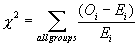A small value of c 2 means the data fit the theoretical distribution well; a large value means they fit the distribution poorly.  This interpretation is straight forward if one looks at the equation for c 2.  If the sample was in fact taken from the theoretical distribution, the number of observations O in each group would be quite close to expected E number in each group.  Therefore the difference between these (O - E) will be quite small.  And the summation shown in the equation would also be quite small.  On the other hand, if the sample came from a different distribution than the theoretical distribution tested, the number of observations expected from the theoretical distribution would be quite different than the number of observations.  This larger difference would lead to a large value of c 2.

Chi-square Goodness-of-Fit Test for Single Independent Sample

ASSUMPTIONS OF CHI-SQUARE GOF TEST FOR SINGLE INDEPENDENT SAMPLE

1)       The sample is a random sample.

2)       The measurement scale is at least nominal.

3)       Each cell contains at least 5 observations (see the discussion under “Trouble shooting” about possible remedies if this assumption is violated).

INPUTS FOR CHI-SQUARE GOF TEST FOR SINGLE INDEPENDENT SAMPLE

The data consist of N independent observations of a random variable X.  These N observations are grouped into c classes (or categories), and the numbers of observations in each class are arranged in the following manner.   Oj is the number of observations in category j, for j = 1, 2, ... , c.

Table 16: Chi- Square Goodness of Fit Table for Single Independent Variable

 Total number of observations Cell/Category/Class Class 1 Class 2 . . . Class j . . . Class c Observed frequency O1 O2 . . . Oj . . . Oc N

HYPOTHESES OF CHI-SQUARE GOF TEST FOR SINGLE INDEPENDENT SAMPLE

Let F (x) be the true but unknown distribution function of X, and let F*(x) be some completely specified distribution function, the hypothesized distribution function.

Ho:  F (x) = F*(x) for all x
Ha:  F (x)
¹ F*(x) for at least one x

The hypotheses may be stated in words.

Ho:  The distribution function of the observed random variable is F*(x).
H
a:  The distribution function of the observed random variable is different than F*(x).

TEST STATISTIC (T ) OF CHI-SQUARE GOF TEST FOR SINGLE INDEPENDENT SAMPLE

Let pj* be the probability of a random observation on X being in category j, under the assumption that F*(x) is the distribution function of X.  Then the expected number of observations in each cell is determined by multiplying the total number of observations N by the probability of each cell pj*.  So Ej is defined as:

Ej = pj*N,        j = 1, 2, . . . , c

where Ej  represents the expected number of observations in class j when Ho is true.  The test statistic T is given by: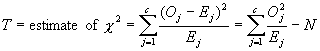INTREPRETATION OF OUTPUT (DECISION RULE) OF CHI-SQUARE GOF TEST FOR SINGLE INDEPENDENT SAMPLE

The approximate distribution of T is the chi-square distribution with (c - 1 - w) degrees of freedom.  Therefore the critical region of approximate size a significance level corresponds to values of T greater than x1- a, the (1 - a) quantile of a chi-square random variable with (c - 1 - w) degrees of freedom, obtained from the Chi-square distribution Table C-2. The analyst rejects Ho if T exceeds x1- a (meaning the two distributions are not alike), otherwise the analyst accepts Ho (meaning the two distributions are alike).

The degree of freedom used is (df = c - 1 - w), where c is the number of categories (or cells) and w is the number of parameters that must be estimated.  The number of parameters that must be estimated w depends on which theoretical distribution you are comparing to the sample.  As an example, suppose one is using this test to determine whether the sample data are compatible with the normal distribution.  Said another way, is the sample data drawn from a parent population having a Normal distribution.  In order to do this, one must estimate the values of the parent population mean (m) and standard deviation (s) by computing the values of the sample data mean (XBAR) and standard deviation (sBAR).  Since in this case two population parameters were estimated from the data, the degrees of freedom would be df = c - 1 - 2 (which requires at least four c categories (cells), since df must be equal to or greater than one).

Chi-square Goodness-of-Fit Test for Two or More Independent Samples

ASSUMPTIONS OF CHI-SQUARE GOF TEST FOR TWO OR MORE INDEPENDENT SAMPLES

1)       Each sample is a random sample.

2)       The outcomes of the various samples are mutually independent (particularly among samples, because independence within samples is part of the first assumption).

3)       Each observation may be categorized into exactly one of the c categories (i.e., the categories are mutually exclusive and collectively exhaustive).

4)       The researcher determines the number of observations in Each sample taken before the data collection phase of a study.

5)       Each cell contains at least 5 observations (see the discussion under “Trouble Shooting” about possible remedies if this assumption is violated).

6)       The measurement scale is at least nominal.

INPUTS FOR CHI-SQUARE GOF TEST FOR TWO OR MORE INDEPENDENT SAMPLES

There are r populations in all, and one random sample is drawn from each population.  Let ni represent the number of observations in the ith sample (from the ith population) for 1 &#8804; i &#8804; r.  Each observation in each sample may be classified into one of c different categories.  Let Oij be the number of observations from the i th sample that fall into class (or category or cell) j, so

ni = Oi1 + Oi2 + . . . + Oij + . . . + Oic       for all i

The data are arranged in the following form, which is called an r x c contingency table.

Table 17: Contingency Table for Chi-Square Goodness of Fit Test
for Two or More Variables

Samples below are drawn from these populations

Class 1

Class 2

. . .

Class
j

. . .

Class c

Totals

Population 1

O1 1

O1 2

. . .

O1 j

. . .

O1 c

n1

Population 2

O2 1

O2 2

. . .

O2 j

. . .

O2 c

n2

. . .

. . .

Population i

O i 1

O i 2

. . .

O i j

. . .

O i c

ni

. . .

. . .

Population r

O r 1

O r 2

. . .

O r j

. . .

O r c

nrTotals

C1

C2

. . .

Cj

. . .

Cc

N

The total number of observations from all samples is denoted by N.  The number of observations in the j th column is denoted by Cj, which is the number of observations from all populations that are in class j.

N = n1 + n2 + ... + ni + ... + nr

Cj = O1j + O2j + . . . + Oij + . . . + Orj       for all j

HYPOTHESES OF CHI-SQUARE GOF TEST FOR TWO OR MORE INDEPENDENT SAMPLES

Let the probability of a randomly selected value from the i th population being classified in the j th class be denoted by pi j, for i = 1, 2, . . . , r, and  j = 1, 2, . . . , c.

Ho:  All of the probabilities in the same column are equal to each other (i.e., p1j = p2j = . . . = prj , for all j).

Ha:  At least two of the probabilities in the same column are not equal to each other (i.e., pi j ¹ pk j for some j, and for some pair i and k).

It is not necessary to stipulate the various probabilities.  The null hypothesis merely states that the probability of being in class j is the same for all populations, no matter what the probabilities might be (and no matter which category is being considered).  This test is sometimes called the “chi-square test for homogeneity” because it evaluates whether or not the r samples are homogeneous with respect to the proportions of observations in each of the c categories.

TEST STATISTIC (T ) OF CHI-SQUARE GOF TEST FOR TWO OR MORE INDEPENDENT SAMPLES

Because two or more samples are being comparing to each other, the expected probabilities of some “known” distribution are not used as a comparison.  Rather, the expected probabilities of each cell, based on what it should be if all distributions are in fact the same (which is our null hypothesis Ho), is calculated.  This is done by calculating the “average” probability of each cell using the sample probabilities.  If in fact all the sample distributions are the same then each cell probability should be close to this average.  If they aren’t the same, then this difference will cause the test statistic to become larger.  Recall that a large test statistic tends to reject the null hypothesis (meaning the analyst rejects the distributions being the same) and accepts the alternative hypothesis (meaning that at least two of the distributions are different).  These “average” expected probabilities Eij , are calculated by: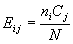Where Ei j represents the expected number of observations in cell (i, j) when Ho is true.  The test statistic T is given by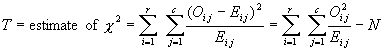INTREPRETATION OF OUTPUT (DECISION RULE) OF CHI-SQUARE GOF TEST FOR TWO OR MORE INDEPENDENT SAMPLES

The approximate distribution of T is the chi-square distribution with (r - )(c - 1) degrees of freedom. Therefore the critical region of approximate size a significance level corresponds to values of T greater than x1- a, the (1 - a) quantile of a chi-square random variable with (r - 1)(c - 1) degrees of freedom, obtained from the Chi-square distribution Table C-2.  The analyst rejects Ho if T exceeds x1-a (meaning the at least two distributions are not alike), other wise she accepts Ho (meaning all the distributions are alike).

Safety Example: In a comparison of formulae for predicting rail-highway crossing hazards (Ardeshir and Demetsky, Transportation Research Record No. 1114, 1987), researchers used four formulae (models) to predict the number of accidents at each of 1,536 rail-highway crossings.  Data and the actual number of observed accidents were available for these 1,536 crossings over a five-year period.  Each formula specifies the distribution of the expected number of accidents over this five-year period.  Therefore, the observed number of accidents can be compared to the expected number of accidents for each model using the chi-square goodness-of-fit test.  Each crossing is treated as a separate category so the chi-square test statistic is computed by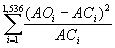where AO is the number of observed accidents and AC is the number of computed accidents at each of the 1,536 crossings.

Using this method, chi-square test statistics were computed for each of the four models separately.  The purpose of this was to compare how well each model fit the actual data.  Or put another way, how well the distribution of accidents obtained from each model fit the distribution of the actual observed

accidents.  The four test statistics were calculated to be to 2176, 3810, 961, and 833.  The authors concluded that the model producing the lowest test statistic was the best fit of the four models.

It should be noted that these authors used the chi-square GOF test to provide information regarding goodness-of-fit of each model compared to each of the other models.  For this purpose, the chi-square test statistics are used as a “measure” of goodness of fit.  No determination was made as to the probability that one or more of the four estimated distributions were statistically likely to be the same as the distribution of the observed data.  To do this, one would obtain the (1 - a) quantile of a chi-square random variable having (r - 1)(c - 1) degrees of freedom.  Since only two distributions are compared at once, r = 2 while c = 1536, resulting in a df = (2 - 1)(1536 - 1) = 1535.  Most statistical table for the chi-square distribution do not list values for df > 100.  However, Conover (1999, p.510) provides a method for estimating these values by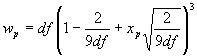where wp is the (1 - a) quantile of a chi-square random variable, df = degrees of freedom, and xp = the value from the standardized normal distribution.  Making these calculations yields values having significance of 0.05 (p-value = 0.95) and 0.01 (p-value = 0.99) of 1627 and 1667 respectively.  Based on these, one could conclude that although there are some deviations between the estimated and observed frequencies in these distributions, the chi-square goodness-of-fit test indicated there is a reasonably high likelihood that the deviations in the two best fitting models (test statistics of 833 and 961) can be attributed to chance alone.  In other words, the analyst is reasonably confident that these two models estimate distributions that are similar to the distributions of the observed data.

Please note that all the assumptions inherent in the chi-square test cannot be verified by the information provided by the authors of this paper.  Specifically, the minimum number of frequencies in each of the 1536 cells is not stated.  This causes concern because accidents are infrequent  occurrences, even when summed over a five year period.  It is recommended that authors specifically state what the underlying assumptions were for all statistical methods used and that they have been met (or if not met, why the method still yields useful information).

Trouble Shooting Chi-square Tests:

How many observations should be included in each category (cell)?

According to Conover (1999, p.241) care must be taken not to let the numerator (Ejs) of the Test statistic T become too small.  If some of the Ejs are small, the asymptotic chi-square distribution may not be appropriate, but just how small is not clear.  Tradition holds that five is the minimum number of expected observations that should be contained in each category (cell).  If a researcher is presented with a situation where less than 5 expected observations occurs in a category, sometimes two or more of the categories can be combined in such a way that none of the Ejs is less than 5.  Using this rule will generally be “safe.”  However, if this is a problem, the researcher is advised to seek more advanced texts like this Chapter’s references.  Research indicates that various relaxations of the general rule may be taken.

Can the number of observations N ever be too large?

In any goodness of fit test Ho will be rejected (indicating the two distributions are different) if the sample size is large enough.  For this reason the Test statistic T is often used as a measure of goodness of fit.  Rejection of the null hypothesis in sufficiently large samples occurs because real data are never really distributed by any theoretical distribution. These theoretical distributions (e.g., normal, Poisson, etc.) are used to approximate real data because of the many properties that can be inferred from a completely known and specified distribution.  What is sought is whether or not the data are “close enough” to a theoretical distribution, so that the theoretical distribution can be used to obtain reasonably accurate results.  A goodness-of-fit test is one way of ascertaining if this agreement is “close enough.”

How can I transform ordinal, interval and ratio scale data to nominal scale data so I can use the chi-square test?

At times, a researcher may want to use the chi-square test for data that have a higher scale than simply nominal.  This means that the researcher is willing to give up the extra power that usually comes from nonparametric tests using ranking methods, such as the Kolmogorov-Smirnov type for ordinal scale or higher data.  To transform ordinal (or interval/ratio) data, one simply has to devise a meaningful scheme to group the data into categories.  There are two practical considerations when doing this.  First, data must be grouped in such a manner that no category has less than five expected occurrences in a category.  The second consideration applies when comparing to a known distribution (say the Normal distribution).  In this case, the categories must be chosen such that the frequencies of the known distribution can be estimated and there are enough categories to insure df ³ 1.  As an example, suppose the following 40 interval scale data points (shown from smallest to largest) are measurements that are suspected of following a normal distribution.

Table 18: Forty Ordered Observations from a Normal Distribution

 1.87 3.65 4.56 5.29 2.32 3.86 4.69 5.29 2.4 3.96 4.71 5.6 2.93 4.05 4.73 5.67 3.01 4.18 4.84 5.72 3.11 4.29 4.86 5.78 3.17 4.29 4.92 5.93 3.34 4.38 5.01 6.2 3.45 4.45 5.01 6.69 3.48 4.55 5.11 7.71

A chi-square test is used, the data are classified into 4 groups.  This will provide us with (c - 1 - w) degrees of freedom, or 1 degree of freedom (4 -1 - 2) since there are four categories, and two parameters will be estimated for the hypothesized normal distribution.

The data are first ordered.  Then the mean is estimated (4.48), and the standard deviation (1.22) of the sample is estimated, to approximate the mean and standard deviation of the hypothesized parent population (now hypothesized to be N (4.48, 1.22)). If groups are created that represent the four quartiles of the N (4.48, 1.22) there will be four groups.  Each group is expected to contain one-fourth of any sample drawn from it.  In this case, that analyst has 40 data points, and so would expect 10 points to be in each quartile.  Each quartile’s range of values can be calculated using the standard normal distribution.  For example, the first quartile will contain values from -¥ to 3.57.  This is calculated using the 0.25 quartile of the standard normal (-0.6725) from the Standard Normal distribution, Table C-1, and transforming it to N (4.48, 1.22).  As the reader will recall, use of the standard normal distribution table requires the “z-transformation” be used which iswhere m is the mean, s the standard deviation, x the data value, and z the transformed value. One computes z (-0.6725), and wants to find x so x = (1.22)(-0.675) + 4.48 = 3.57.  Therefore, the values for the first quartile will be -¥ £ x £ 3.57.  In similar fashion, the other quartile cut points can be calculated as 4.48, 5.30, and +¥.  Separating the observations using these boundaries results in four groups, each containing the measurements that would be in the appropriate quartile assuming they are distributed N (4.48, 1.22).

Table 19: Forty Normal Observations Binned into Quartiles

 1st quartile 2nd quartile 3rd quartile 4th quartile 1.87 3.65 4.55 5.60 2.32 3.86 4.56 5.67 2.40 3.96 4.69 5.72 2.93 4.05 4.71 5.78 3.01 4.18 4.73 5.93 3.11 4.29 4.84 6.20 3.17 4.29 4.86 6.69 3.34 4.38 4.92 7.71 3.45 4.45 5.01 3.48 5.01 5.11 5.29 5.29 no. observations 10 9 13 8 range -¥ £ x £ 3.57 3.57 £ x £ 4.48 4.48 £ x £ 5.30 5.30 £ x £ +¥

The analyst can now use the chi-square test for a single independent sample, which is described in the next section.  For the 4 categories, the observed frequencies will be 10, 9, 13, and 8 while the expected frequencies assuming a theorized N (4.48, 1.22) distribution will be 10, 10, 10, and 10. More categories could have been used. Assuming that categories of equal probability size were used, then the largest number of categories is eight, which still maintains a minimum of five expected occurrences in each category.

Goodness-of-Fit Tests for Ordinal, Interval, and Ratio Scale Data - Kolmogorov-Smirnov Type Tests

Kolmogorov and Smirnov developed procedures which compare the empirical distribution functions (EDF) of two samples to see if they are similar--that is, drawn from the same known or unknown parent population. The empirical distribution function (EDF) is a cumulative probability distribution function constructed from observed or experimental data (see definition of EDF for more detailed information).  Two cumulative functions can be compared graphically, which has an innate appeal, but Kolmogorov and Smirnov developed more rigorous statistical procedures that are discussed in this section.  All these procedures use the maximum vertical distance between these functions of how well the functions resemble (fit) each other--or said another way, their “goodness of fit.”

Kolmogorov developed statistics that are functions of the maximum vertical distance between an EDF S (x) of an unknown distribution and the cumulative distribution function CDF F (x) of a known distribution.  These are one-sample tests and are said to be of the Kolmogorov-type.  Smirnov worked with these same maximum distances but between two empirical density functions.  These types of statistics are called the Smirnov-type.  All Kolmogorov-Smirnov type tests are for continuous distributions whereas the chi-square is valid for both continuous and discrete distributions.  However, one may still use the Kolmogorov-Smirnov type tests for discrete distributions realizing the results yield a conservative approximation for the critical levels. They are often preferred over the chi-square GOF test if the sample size is small.  The chi-square test assumes the number of observations is large enough so that this distribution provides a good approximation of the distribution of the test statistic, whereas the Kolmogorov test is exact even for small samples.  Also the Kolmogorov-Smirnov type tests are more efficient with data and usually more powerful.

Kolmogorov introduced his GOF test for a single sample in 1933.  It provides an alternative to the chi-square GOF test when dealing with data of ordinal scale or higher.

Smirnov introduced his GOF test for two samples in 1939.  It is similar to the Kolmogorov single sample test except it compares two unknown empirical distribution functions rather than an unknown EDF to a known CDF.  It is important to note that much of the literature refers to these tests by combining the names of two originators and distinguishing them by the number of samples; after this fashion, the tests are called the Kolmogorov-Smirnov GOF test for a single sample and the Kolmogorov-Smirnov GOF test for two samples.

Kolmogorov Goodness-of-Fit Test for Single Independent Sample (Ordinal, Interval, and Ratio Scale Data)

ASSUMPTIONS OF KOLMOGOROV GOF TEST FOR SINGLE INDEPENDENT SAMPLE

1)       The sample is a random sample.

2)       The measurement scale is at least ordinal.

3)       The theoretical distribution (and by implication the sample distribution) is continuous.  Test may still be used for discrete distributions but doing so leads to a conservative test.  If this is not adequate, Conover (1999, p.435-437) details a method of obtaining the exact critical level for a discrete distribution.

4)       All parameters of the hypothesized distribution function are known, that is the distribution function is completely specified.  If parameters have to be estimated from the sample, the test becomes conservative.

INPUTS FOR KOLMOGOROV GOF TEST FOR SINGLE INDEPENDENT SAMPLE

The data consist of n independent observations of a random variable X1, X2, . . . , Xn, associated with some unknown distribution function F (x).

HYPOTHESES OF KOLMOGOROV GOF TEST FOR SINGLE INDEPENDENT SAMPLE

Let F (x) be the true but unknown distribution function of X, and let F*(x) be some completely specified distribution function, the hypothesized distribution function.

A.  Two-sided test

Ho:  F (x) = F*(x) for all x
Ha:  F (x)
¹ F*(x) for at least one x

B.  One-sided test

Ho:  F (x) ³ F*(x) for all x
Ha:  F (x) < F*(x)
for at least one x

This is used when the distributions are suspected of being the same except that the sample distribution is shifted to the right of the hypothesized distribution.  In other words, the values of the F (x) tend to be larger than the values of the F*(x).  This is a more general test than testing for the distributions only differing by a location parameter (means or medians).

C.  One-sided test

Ho:  F (x) £ F*(x) for all x
Ha:  F (x) > F*(x)
for at least one x

This is the one-side test to use when the distributions are suspected of being the same except that the sample distribution is (smaller) shifted to the left of the hypothesized distribution.

TEST STATISTIC (T, T +, T -) OF KOLMOGOROV GOF TEST FOR SINGLE INDEPENDENT SAMPLE

Let S (x) be the the empirical distribution function (EDF) based on the random sample X1, X2, . . . , Xn.  The test statistic T is defined differently for hypotheses sets A, B and C.  The graph gives a general illustration of the two distribution functions being compared, showing how T, T+ and T- are obtained.

A.  Two sided test:  The test statistic atistic T is the maximum (denoted by “sup” for supremum) vertical difference between S(x) and F*(x):B.  One-sided test:  The test statistic T+ is the maximum vertical difference by F*(x) above S(x):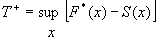C.  One-sided test:  The test statistic T-  is the maximum vertical difference by S(x) above F*(x):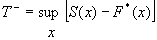Figure 21: Comparison of Two Distribution Functions

INTREPRETATION OF OUTPUT (DECISION RULE) OF KOLMOGOROV GOF TEST FOR SINGLE INDEPENDENT SAMPLE

Reject the null hypothesis Ho at the level of significance a (meaning the two distributions are not alike) if the appropriate test statistic (T, T+ or T-) exceeds the 1 - a quantile (w1 - a) as given in the Table C-10, otherwise accept Ho (meaning the two distributions are alike).  Note that the two-sided test statistic T is always equal to the larger of the one-sided test statistics T+ and T-.

Conover (1999, p.438-439) details one of the most useful features of the Kolmogorov two-sided test, the ability to form a confidence band for the true unknown distribution function F (x).  This allows upper and lower bounds to be placed on the graph of the EDF S (x) and make the statement that the unknown distribution function F (x) lies entirely within those bounds, with 1- a confidence that the statement is correct.  The reader is directed to the reference for more details and the methodology.

Environment Example:  In a reexamination of previous papers that fitted air quality data to hypothetical probability distributions (Chock and Sluchak, Atmospheric Environment, V.20, N.5, 1986), the researchers built the case that the six goodness of fit (GOF) methods used gave varying results as to which of the six hypothesized parent distributions the samples were drawn from.  Air quality data are frequently fitted to hypothetical probability distributions--in this case to lognormal, gamma, beta, Weibull and Johnson distributions--with the intent of using the distributions to predict the number of days on which observed ozone and carbon monoxide concentrations exceed National Ambient Air Quality Standards.  Since these GOF methods did not agree, and for other reasons stated by the researchers, they conclude that “best” fitted distributions of air quality data should not be used to predict the very extreme values used in setting air quality standards.  They suggest that a less-extreme standard, such as using the 95th percentile of the data, would significantly reduce the impact of the intrinsic uncertainty of the estimate.

Only three of the six GOF methods used are statistical GOF methods capable of supporting hypothesis testing: chi-square, Kolmogorov-Smirnov, and Cramer-von Mises-Smirnov.  In reporting the results, the significance for the chi-square GOF test is given.  However, for the other two tests the significances are not reported or is any hypothesis testing done.  The researchers only use qualitative statements such as “fit the . . . data set well.”  It is recommended that when possible, and it is certainly possible with statistical GOF tests, a hypothesis test be done at a stated significance level or a p-value be reported to allow the reader to adequate evaluate the reported results.

While the six GOF tests do vary in selecting the best fitting distribution, it is interesting to note that the three statistical GOF tests all did yield the same result.  These three methods--Chi-square, Kolmogorov-Smirnov, and Cramer-von Mises-Smirnov--all picked the same distribution of those offered as the best fitting for the two samples.  They did, however, pick different distributions for each of the two samples.  The three other GOF tests employed by the researchers--absolute deviation, weighted absolute deviation, and log-likelihood value-- gave varying results.

The two samples used by the researchers to support their arguments were actually the same data.  The first sample had 122 data points and the second sample was 120 of these points, the two removed points being the lowest values, deemed to be outliers by the authors.  The best fitting hypothetical distributions for the two samples differed.  This illustrates the importance of “outliers.”  It is recommended that “outliers” never be removed from data without good justification.  Furthermore, that justification should be stated in the findings--as the authors did in this example paper.  Obviously, the removal of these two “outliers” had a significant impact on the conclusions drawn in this paper and interested readers would want to know the reasons for removing the two outliers so they can form their own conclusions.

It should be noted once again the importance of specificity when discussing statistical tests used to reach conclusions--either by citing a reference for the statistical test(s) used or by reporting sufficient information such that the reader can accurately deduce the specific test statistic/method used.  In this example the researchers list the results for the “Kolmogorov-Smirnov statistic” GOF test for each of the six distributions tested.  The values range from about 5.5 to 9.5.  The Kolmogorov GOF test described in this manual uses the maximum “vertical distance” between the empirical distribution function (EDF) of the data and the cumulative distribution function (CDF) of the hypothesized distribution as the test statistic.  This means that the test statistic ranges from 0.0 to 1.0.  Obviously the researchers were using some other form of a Kolmogorov-Smirnov type test statistic and a compatible set tables to interpret the results.  Again, researchers are cautioned not to use the test statistics given in this manual with tables taken from some other source unless certain that they are compatible.  The researchers did cite a reference for the Cramer-von Mises-Smirnov GOF test they used.  This test was devised by the three statisticians for whom it is named between 1928 and 1936.  The citation given by the researchers was a 1946 book by Cramer.  While this documents the test adequately, such an old reference may be generally inaccessible to most readers.  Thought should be given to citing more current references to assist the interested reader.

Additional Nonparametric GOF Tests For Single Independent Sample (Ordinal, Interval, and Ratio Scale Data)

LILLIEFORS GOF TESTS FOR NORMAL AND EXPONENTIAL DISTRIBUTIONS FOR SINGLE INDEPENDENT SAMPLE

The Kolmogorov GOF test assumes the theorized distribution is completely specified, i.e., no parameters have to be estimated from the sample.  If parameters are estimated from the sample, then it becomes conservative.  To provide more precise tests, additional tables have been developed using the same Kolmogorov test statistic.  The tables vary for the specific hypothesized distribution being tested.  These tests are still nonparametric because the validity of the test (the a level) does not depend on untested assumptions regarding the population distribution.  Rather, the population distribution form is the hypothesis being tested.

Lilliefors developed a GOF test in 1967, which tests the composite hypothesis of normality.  Under this method, the null hypothesis states that the sample is drawn, not from a single specified distribution, but from the family of normal distributions.  This allows that neither the mean nor variance of the normal distribution must be specified.  Conover (1999, p.443-447) provides the detailed method and table for this test.  In a similar manner, Lilliefors later (1969) developed a GOF test for the family of exponential distributions.  This test method and tables are also detailed in Conover (1999, p.447-449).  The power of both these tests are believed to be greater than the chi-square test.  A potentially useful application of the Lilliefors GOF test for exponential distributions is when a researcher is theorizing that when events occur randomly, the time between events follow an exponential distribution.  In this situation, the test can be used as a test of randomness of the data.

SHAPIRO-WILK GOF TEST FOR NORMAL DISTRIBUTION FOR SINGLE INDEPENDENT SAMPLE

Another test for normality of an EDF is the Shapiro-Wilk GOF test.  Some studies have concluded that this test has greater power than the Lilliefors test in many situations.  This test is not of the Kolmogorov-type.  Conover (1999, p.450-451) provided the detailed method and tables for this test.  A useful feature of this test is highlighted though an example by Conover, wherein several independent goodness-of-fit tests are combined into one overall test of normality.  This allows several small samples from possibly different populations, which by themselves are insufficient to reject the hypothesis of normality, to be combined and thereby provide enough evidence to disprove normality.

Smirnov Goodness-of-Fit Test for Two Independent Samples (Ordinal, Interval, and Ratio Scale Data)

ASSUMPTIONS OF SMIRNOV GOF TEST FOR TWO INDEPENDENT SAMPLES

3)       The samples are random samples.

4)       The two samples are mutually independent.

5)       The measurement scale is at least ordinal.

6)       The theoretical distribution (and by implication the sample distribution) is continuous.  May still be used for discrete distributions but doing so leads to a conservative test.

INPUTS FOR SMIRNOV GOF TEST FOR TWO INDEPENDENT SAMPLES

The data consist of two independent random samples, one of size n, X1, X2, . . . , Xn, associated with some unknown distribution function F (x).  The other sample is of size m, Y1, Y2, . . . , Ym, associated with some unknown distribution function G (x).

HYPOTHESES OF SMIRNOV GOF TEST FOR TWO INDEPENDENT SAMPLES

A.  Two-sided test

Ho:  F (x) = G (x)            for all x
Ha:  F (x)
¹ G (x)            for at least one x

B.  One-sided test

Ho:  F (x) £ G (x) for all x
Ha:  F (x) > G (x)
for at least one x

This is used when the distributions are suspected of being the same except that the sample distribution F (x) is shifted to the left of the sample distribution G (x).  In other words, the X values of the F (x) tend to be smaller than the Y values of the G (x).  This is a more general test than testing for the distributions only differing by a location parameter (means or medians).

C.  One-sided test

Ho:  F (x) ³ G (x) for all x
Ha:  F (x) < G (x)
for at least one x

This is the one-side test to use when the distributions are suspected of being the same except that the sample distribution F (x) (X values) is (larger) shifted to the right of the sample distribution G (x) (Y values).

TEST STATISTIC (T, T +, T -) OF SMIRNOV GOF TEST FOR TWO INDEPENDENT SAMPLES

Let S1 (x) be the empirical distribution function (EDF) based on the random sample X1, X2, . . . , Xn. Let S2x) be the empirical distribution function (EDF) based on the other random sample Y1,Y2,. . , Ym.  The test statistic T is defined differently for hypotheses sets A, B and C.

A.  Two sided test:  The test statistic T is the maximum difference between the two EDFs, S1(x) and S2(x):B.  One-sided test:  The test statistic T+ is the maximum difference by S1(x) above S2(x):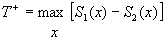C.  One-sided test:  The test statistic T-  is the maximum difference by S2(x) above S1(x):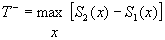INTREPRETATION OF OUTPUT (DECISION RULE) OF SMIRNOV GOF TEST FOR TWO INDEPENDENT SAMPLES

Reject the null hypothesis Ho at the level of significance a (meaning the two distributions are not alike) if the appropriate test statistic (T, T+ or T-) exceeds the 1 - a quantile (w1 - a) as given in the Table C-11 of n = m or Table C-12 if n ¹ m, otherwise accept Ho (meaning the two distributions are alike). Note that the two-sided test statistic T is always equal to the larger of the one-sided test statistics T+ and T-.

Additional Nonparametric GOF Tests For Several Independent Samples (Ordinal, Interval, and Ratio Scale Data)

BIRNBAUM-HALL TEST FOR THREE INDEPENDENTSAMPLES

This test is an extension of the two-sided Smirnov test, which may be used for any number of equal size samples.  However, tables for three samples are all that are readily available in the reference given.  Similar to finding the maximum distance between S1 (x) and S2 (x) for the Smirnov test, the Birnbaum-Hall test find the maximum distance that exists between any combination of two of the three EDFs being tested: S1 (x), S2 (x), S3 (x).  Conover (1980, p.377-379) details the methodology for this GOF test for Ordinal scale or better data and includes the necessary table for finding the critical values for three samples.  However, Conover omits this test in the third edition of his book (1999) because most data sets are not of equal size, a requirement of this test.  For multiple samples of unequal size, the researcher can use the nonparametric Chi-square GOF test for two or more independent samples.

ONE-SIDED AND TWO-SIDED SMIRNOV TESTS FOR THREE OR MORE INDEPENDENT SAMPLES

These tests are similar to the Birnbaum-Hall tests but tables are available from Conover (1980, p.379-384) for up to 10 samples, which must all be of the same size.  Again however, Conover (1999) omits these tests in the third edition of his book (1999) because most data sets are not of equal size, a requirement of these tests.  The Chi-square GOF test for two or more independent samples can be used when dealing with unequal sample sizes.